# Equation Pom Pom Hats

23 products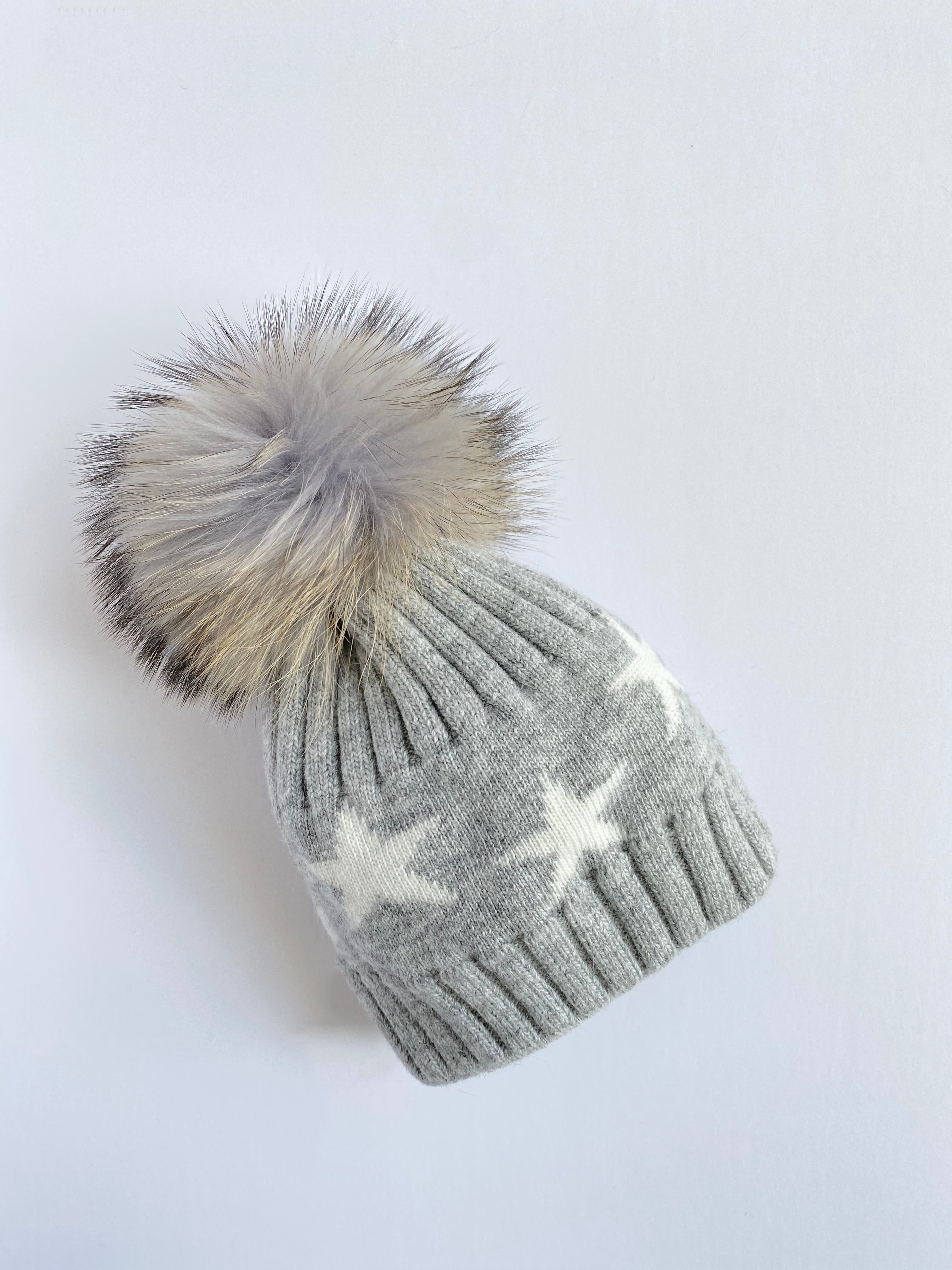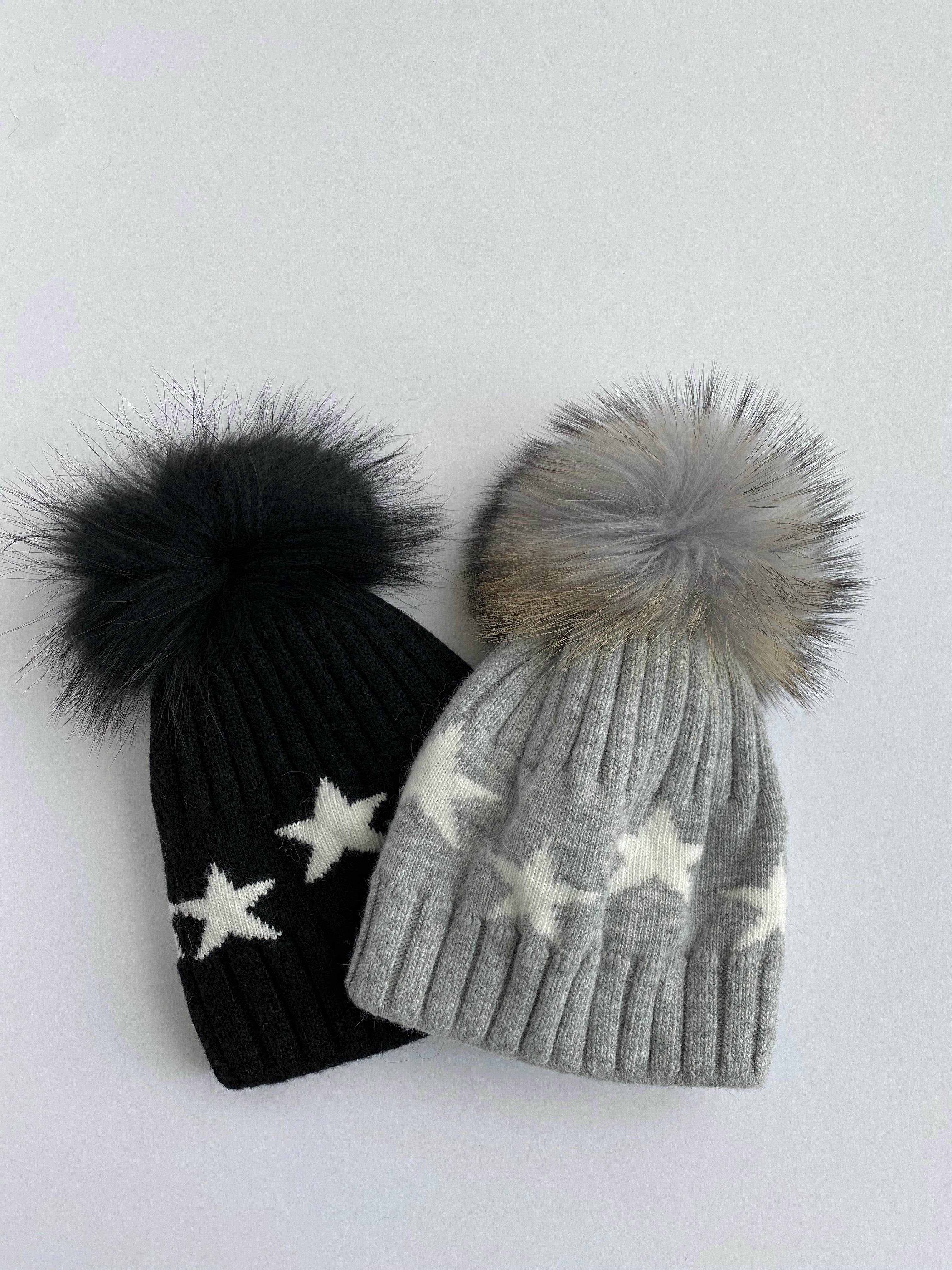Equation Starry Hat in Heather Gray with white stars
EQUATION
\$65.00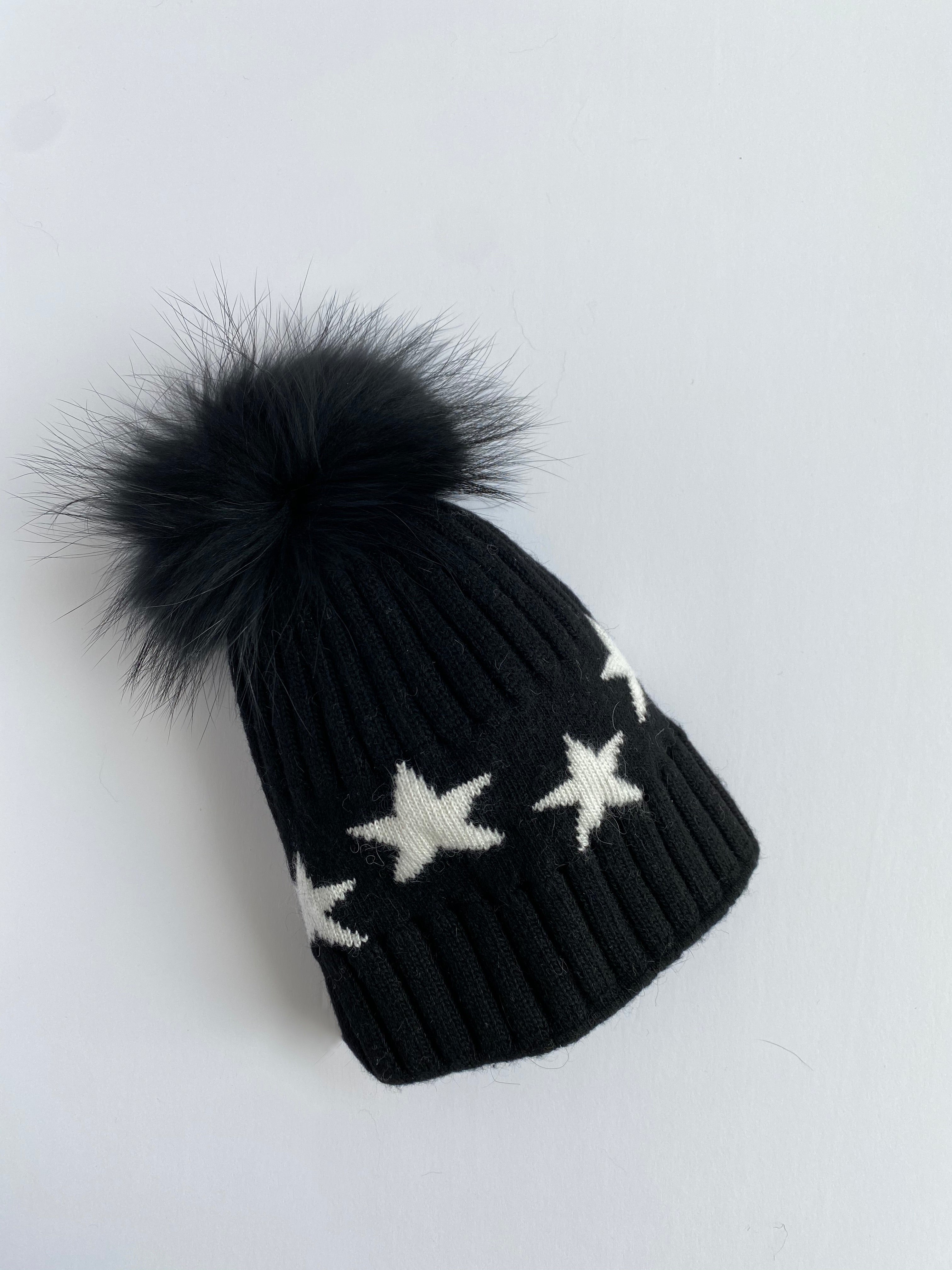Equation Starry Hat in Black with white stars
EQUATION
\$65.00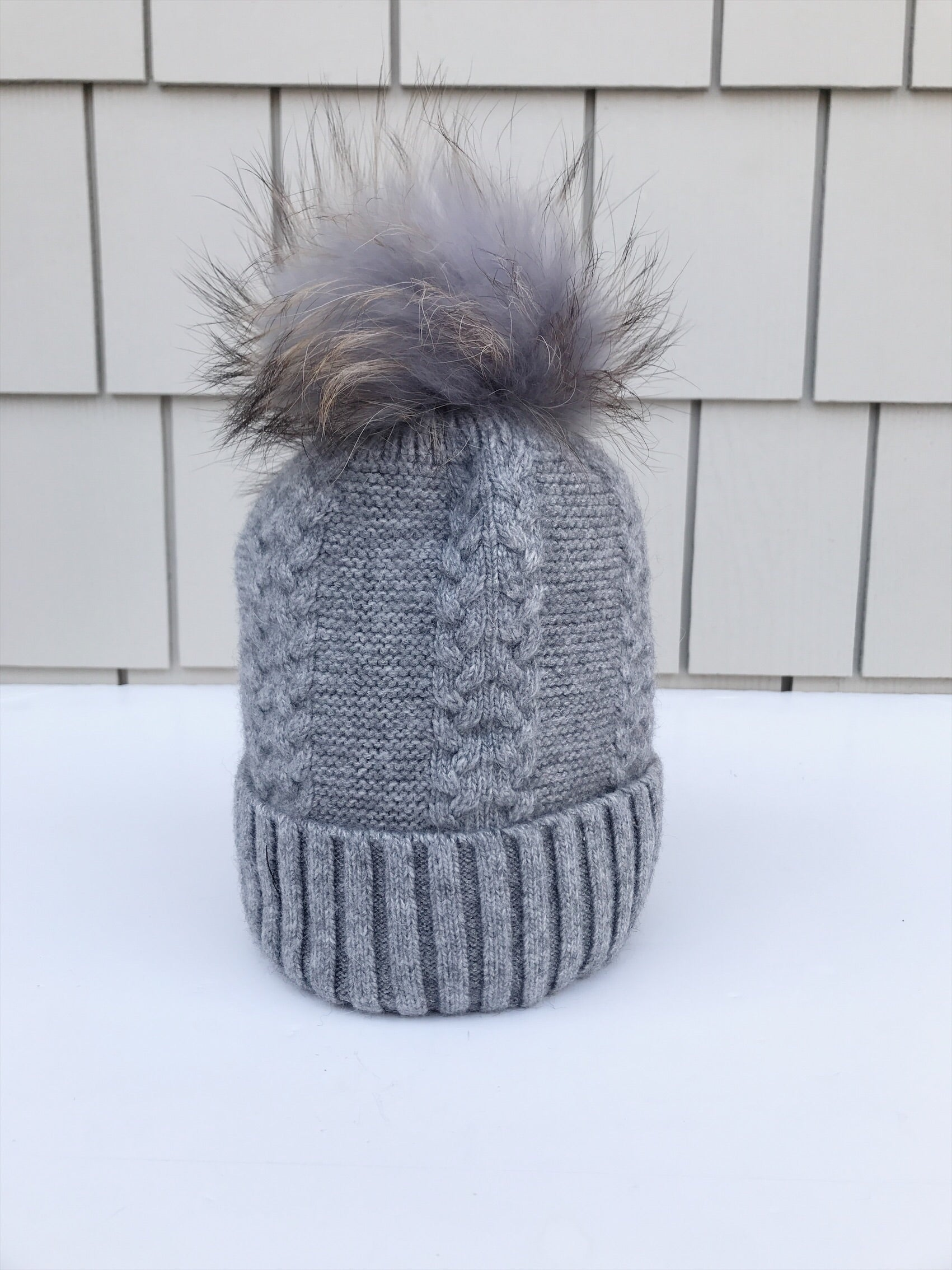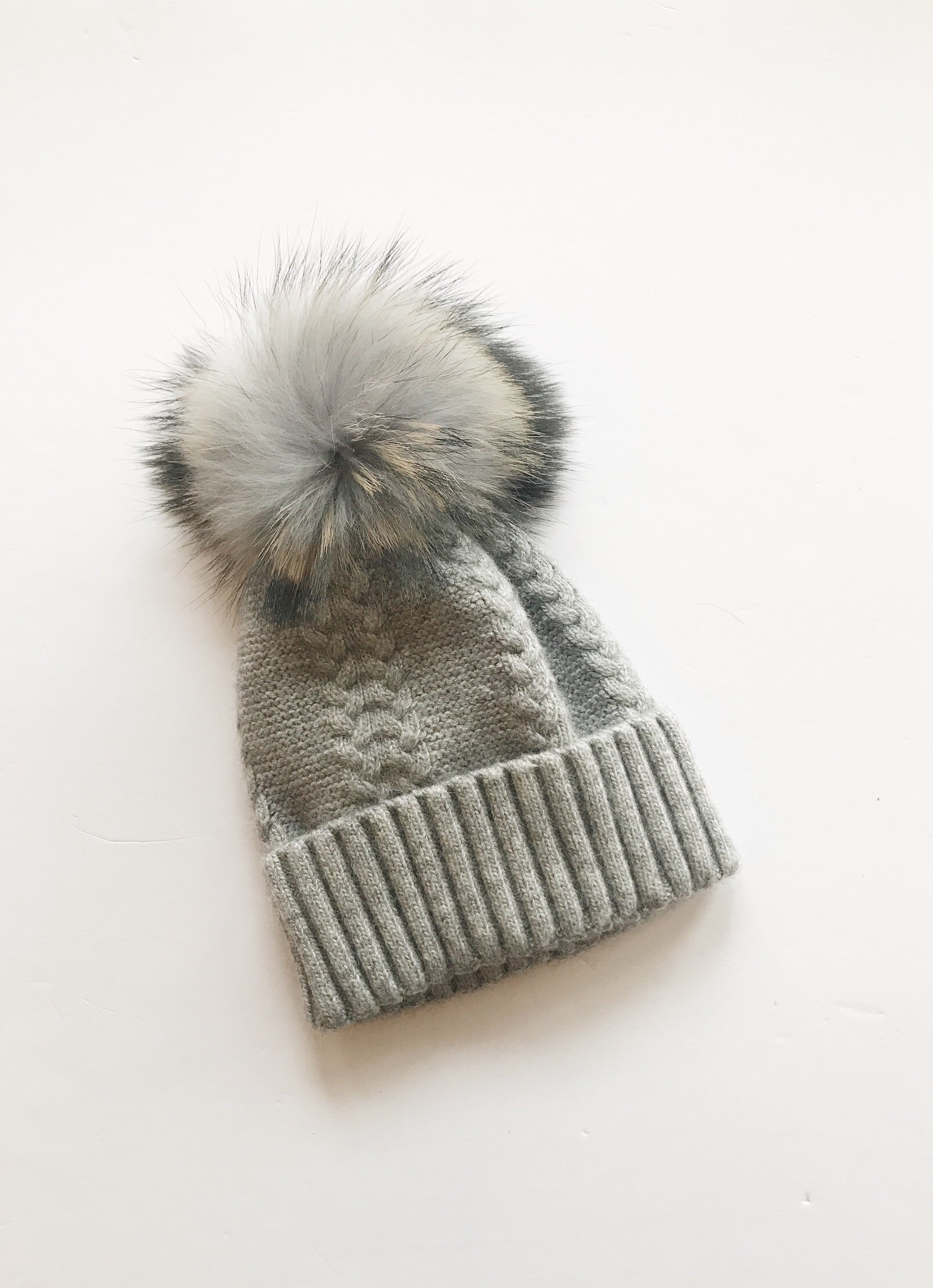Equation Olivia Hat in Gray w/ Gray pom
EQUATION
\$65.00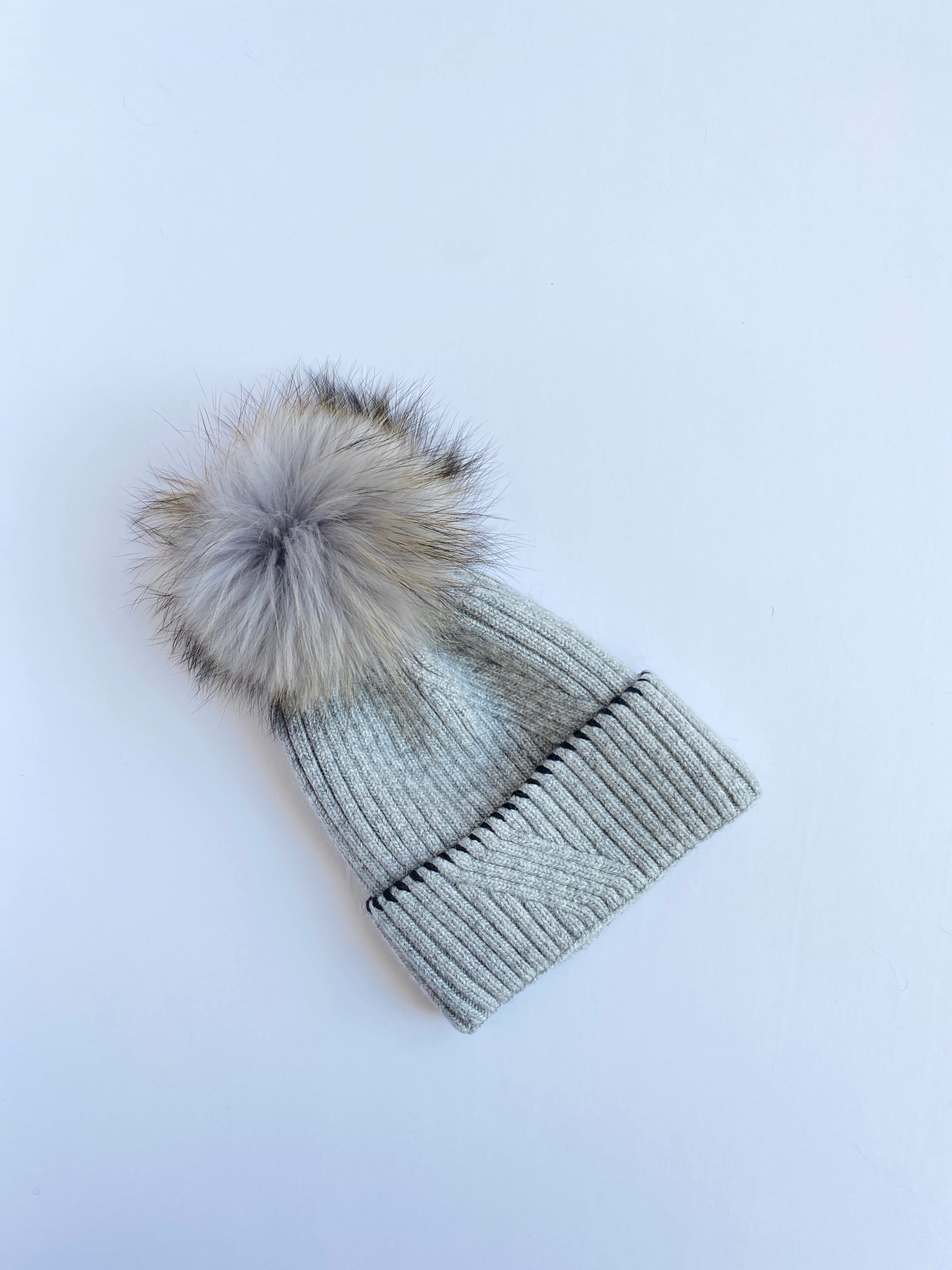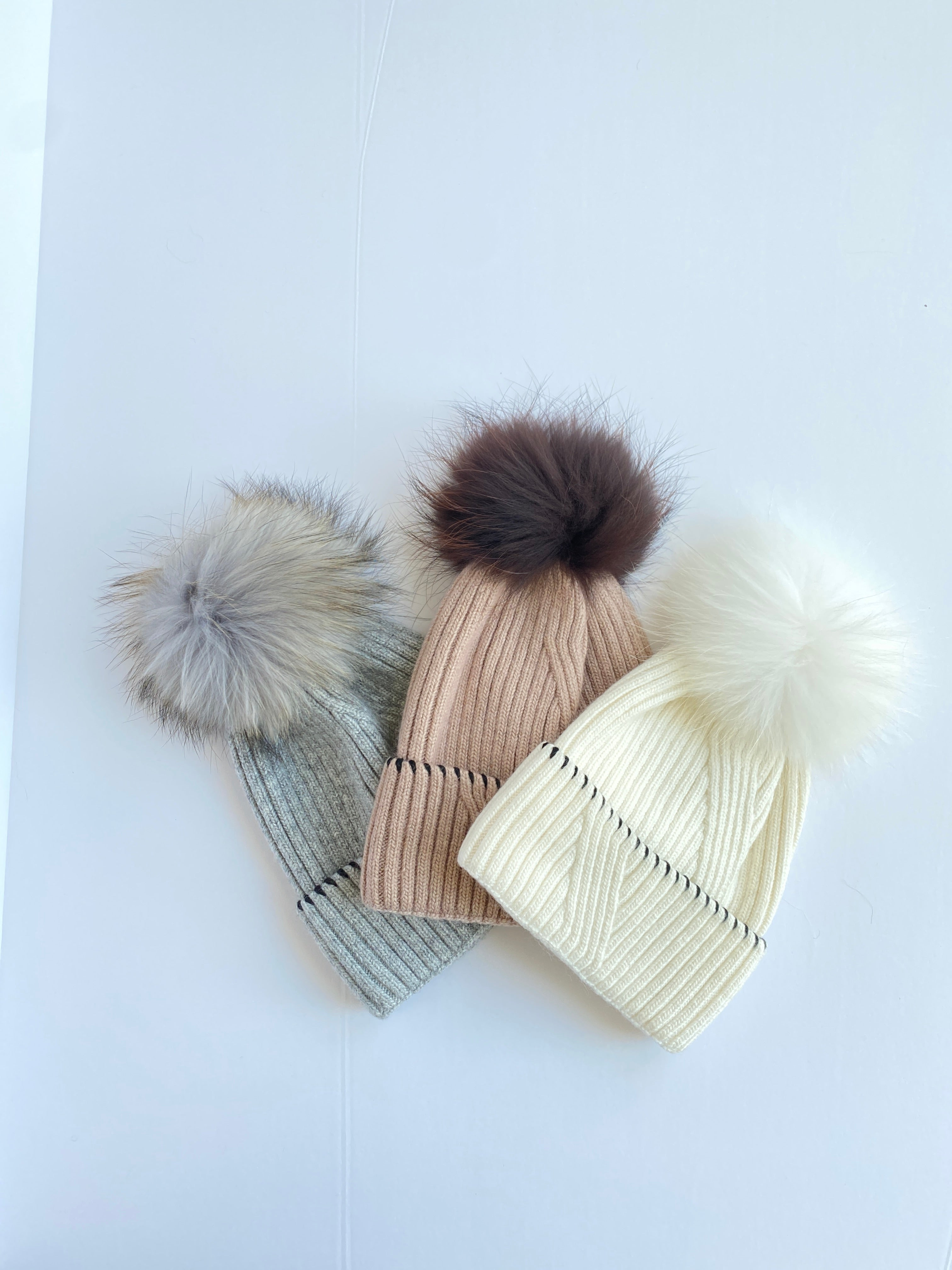Equation Kirstin Hat in Gray
EQUATION
\$70.00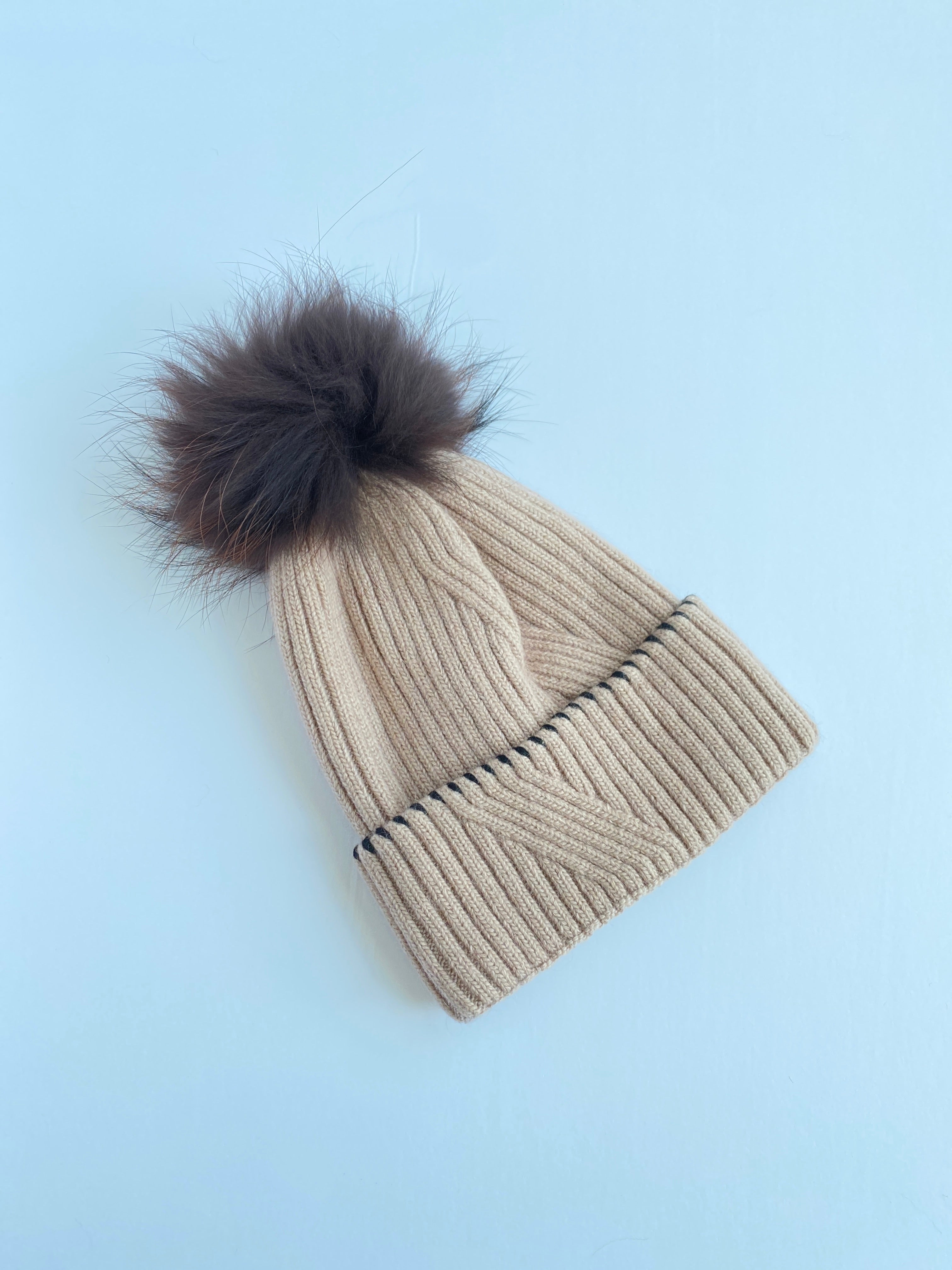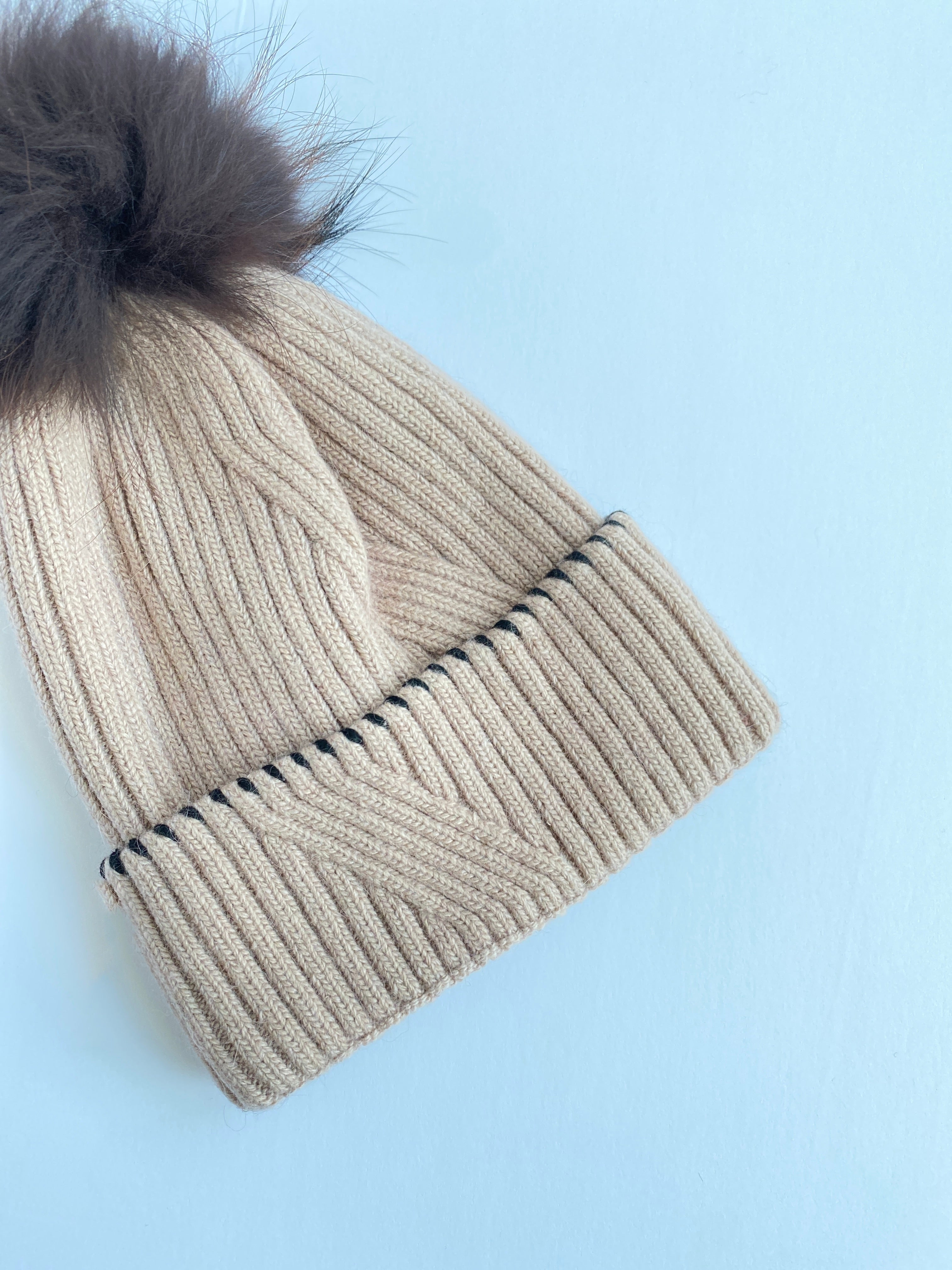Equation Kirstin Hat in Light Brown
EQUATION
\$70.00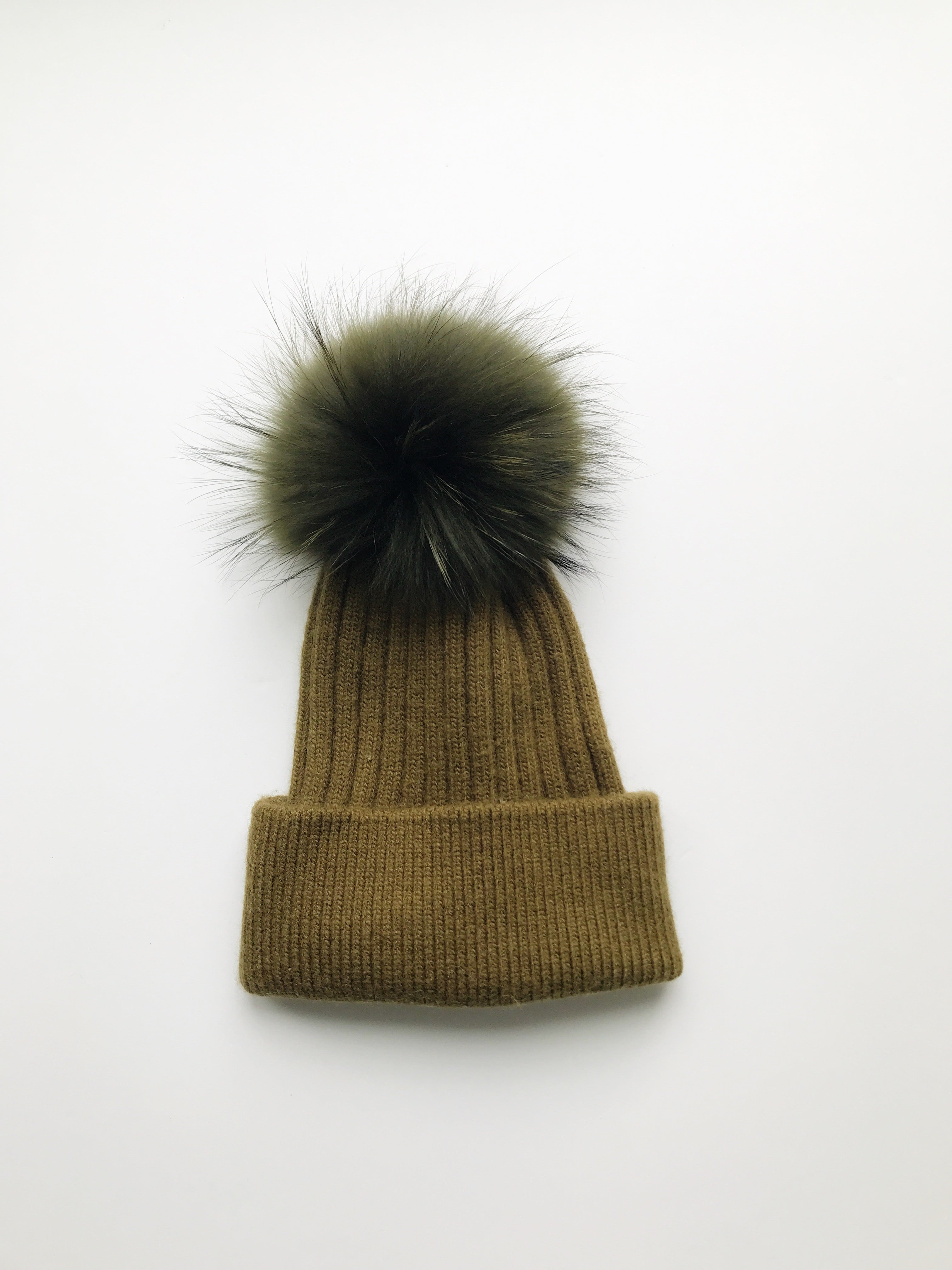Equation Elliott Hat in Olive w/ Olive pom
EQUATION
\$65.00Equation Milla Hat in White/Gray
EQUATION
\$65.00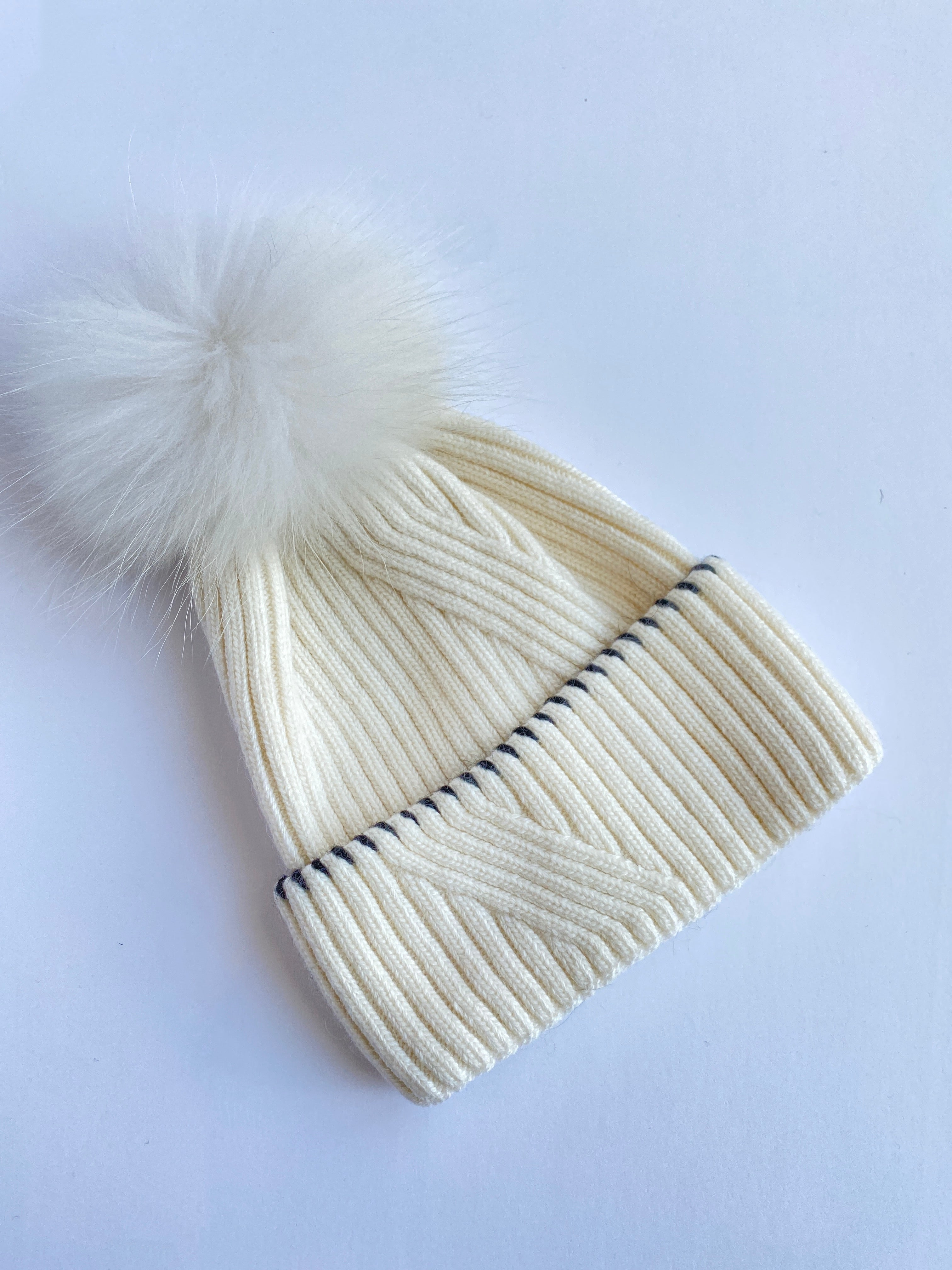Equation Kirstin Hat in Cream
EQUATION
\$70.00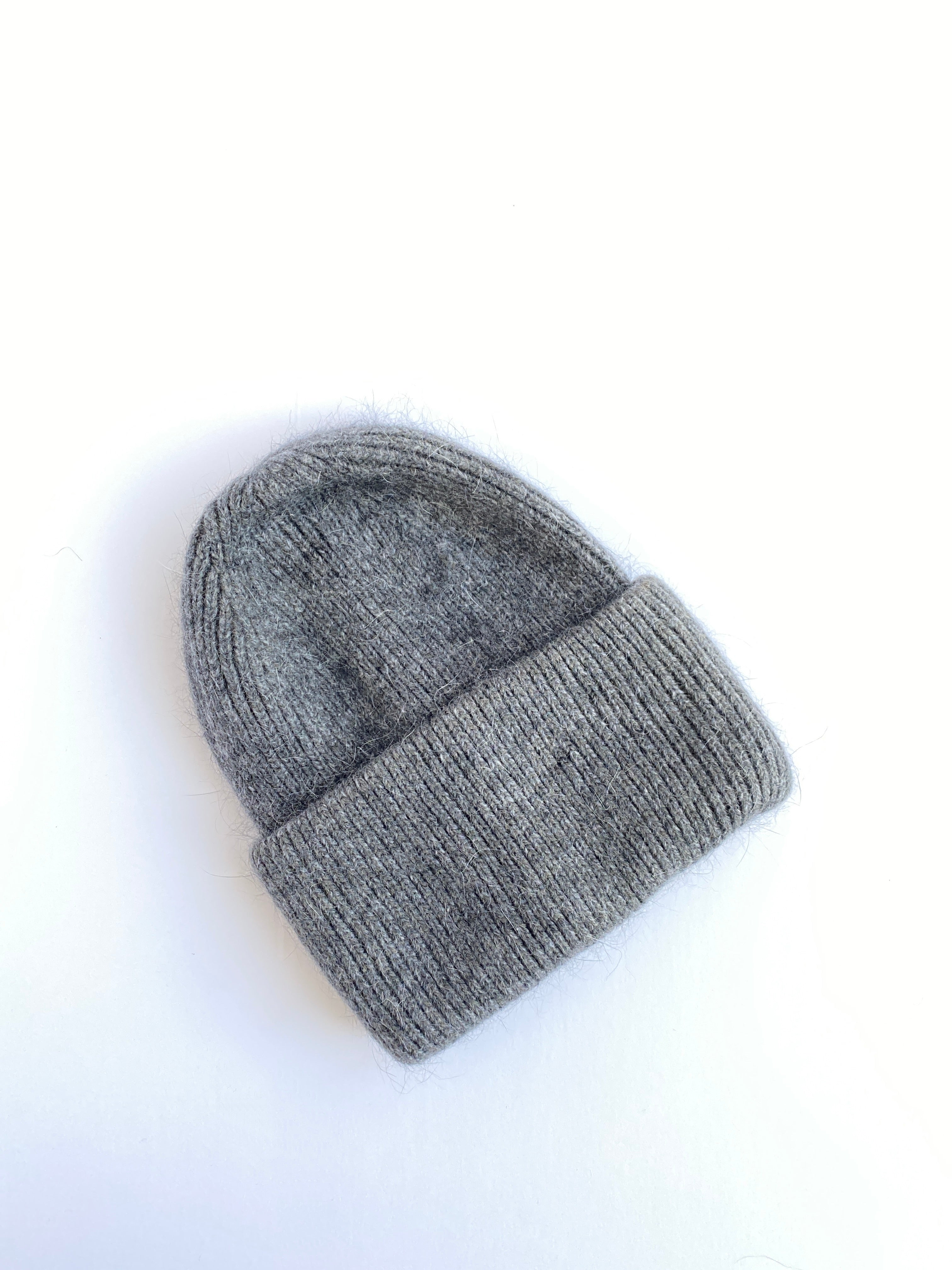Equation SAM Hat in Gray
EQUATION
\$50.00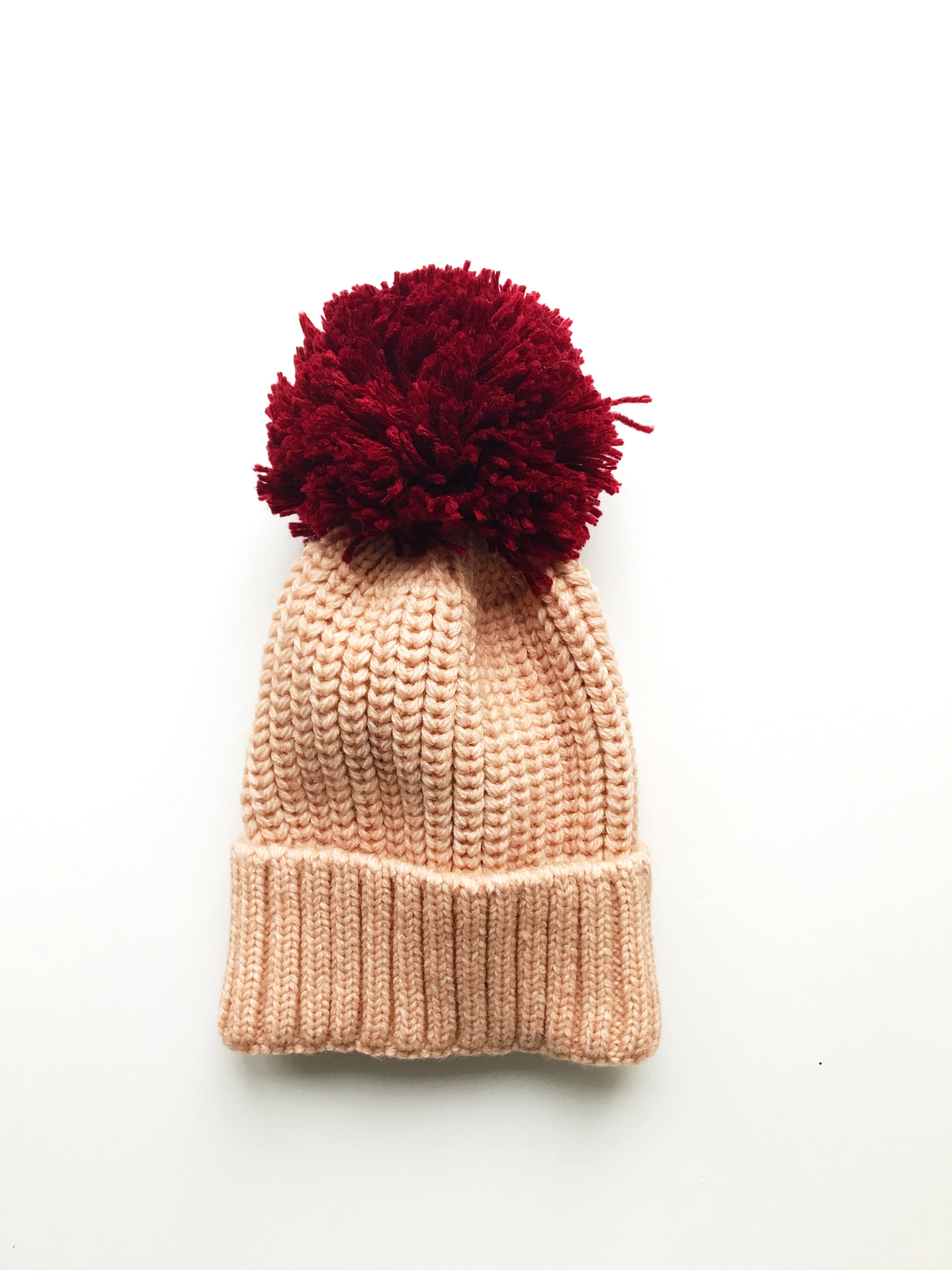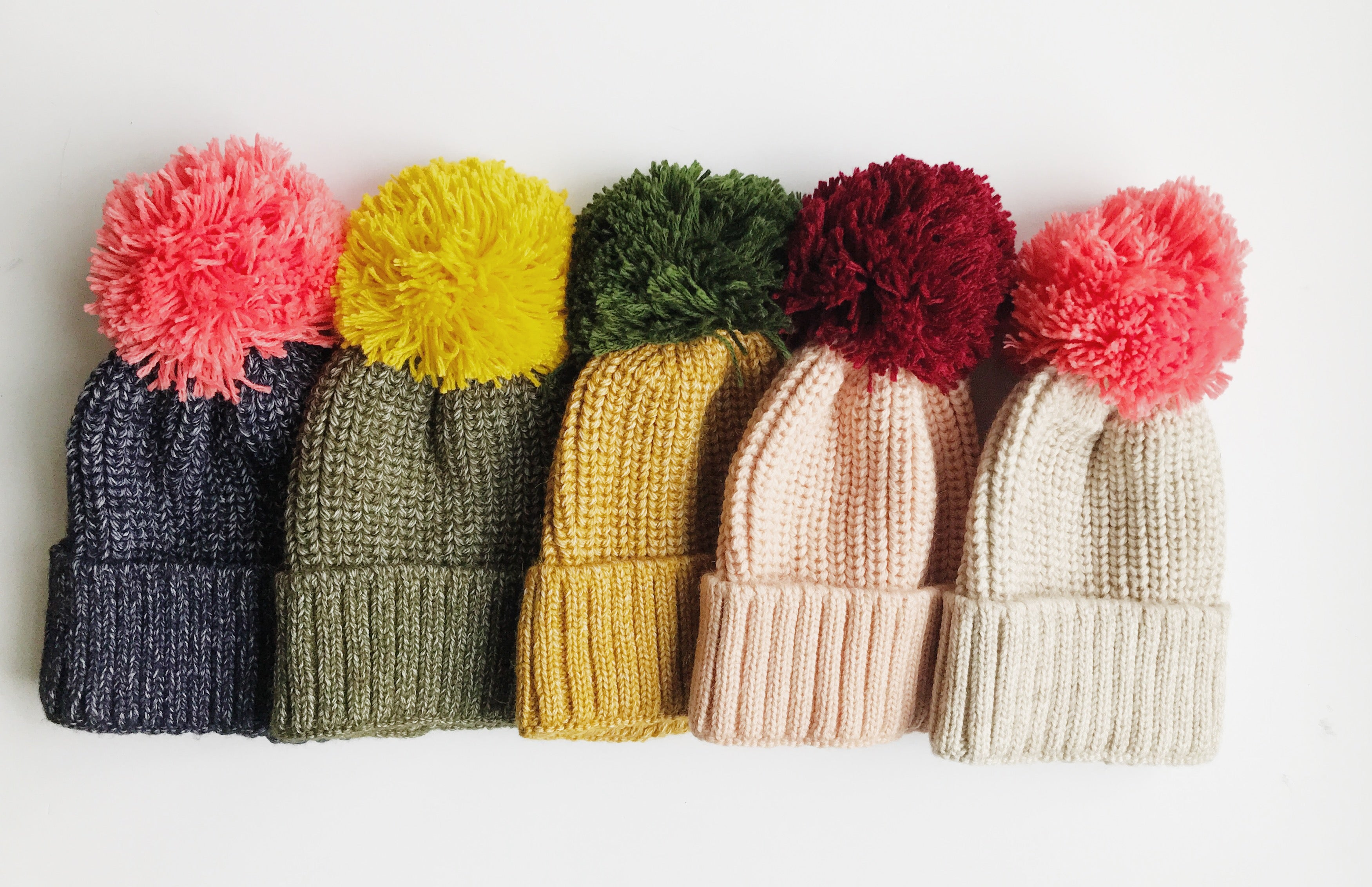Equation Penelope Beanie Hat
EQUATION
\$58.00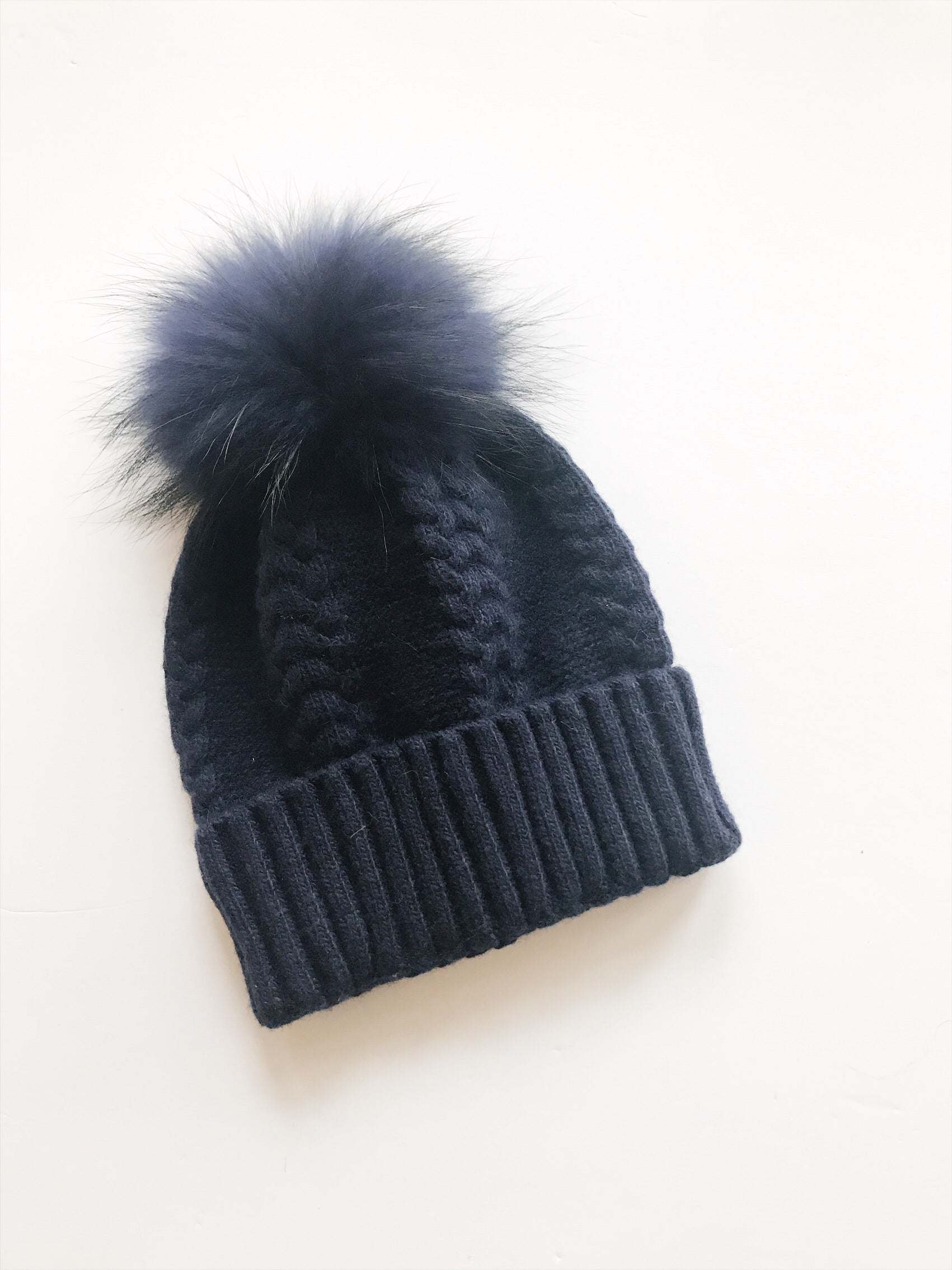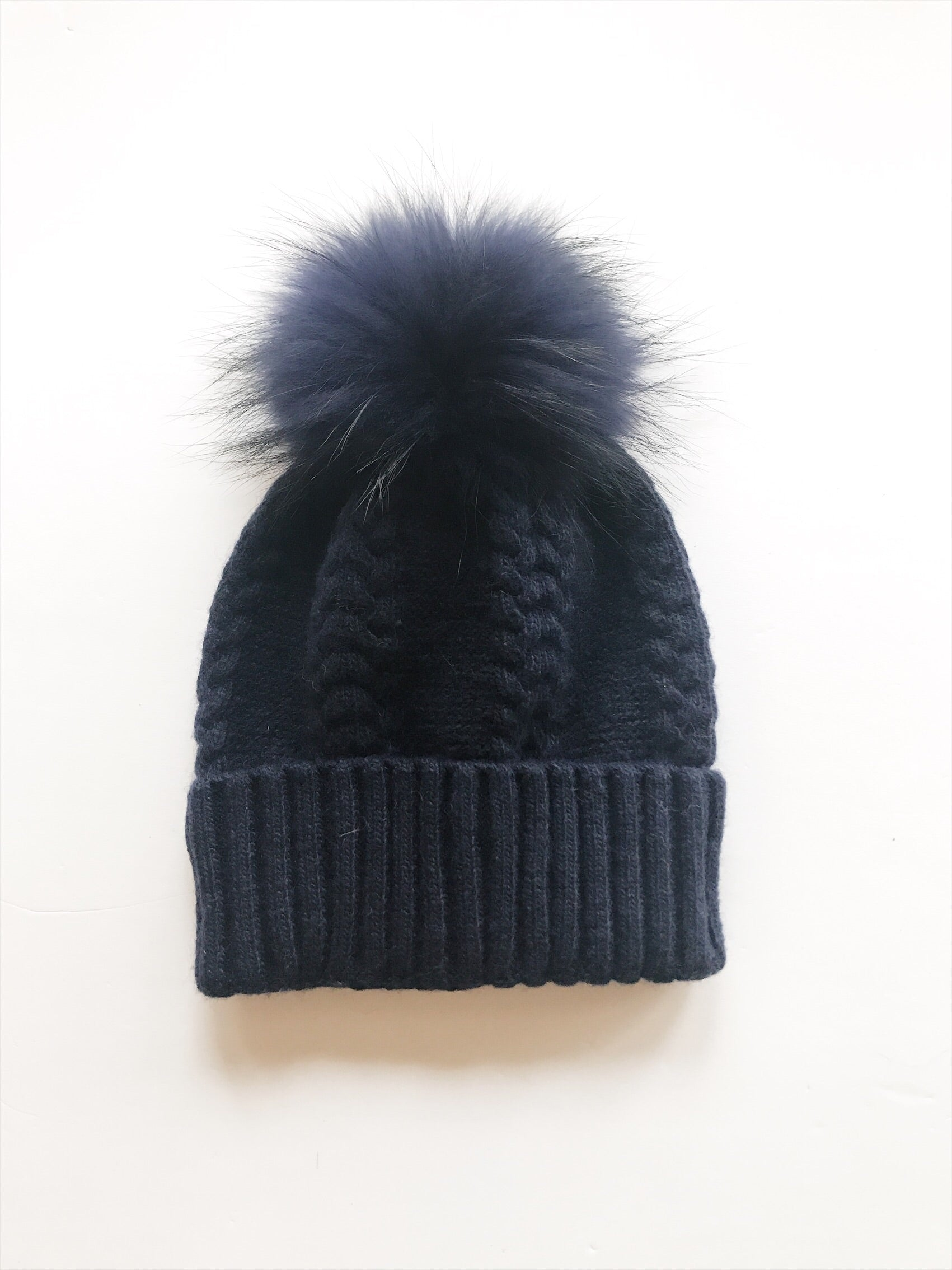Equation Olivia Hat in Navy w/ Navy pom
EQUATION
\$65.00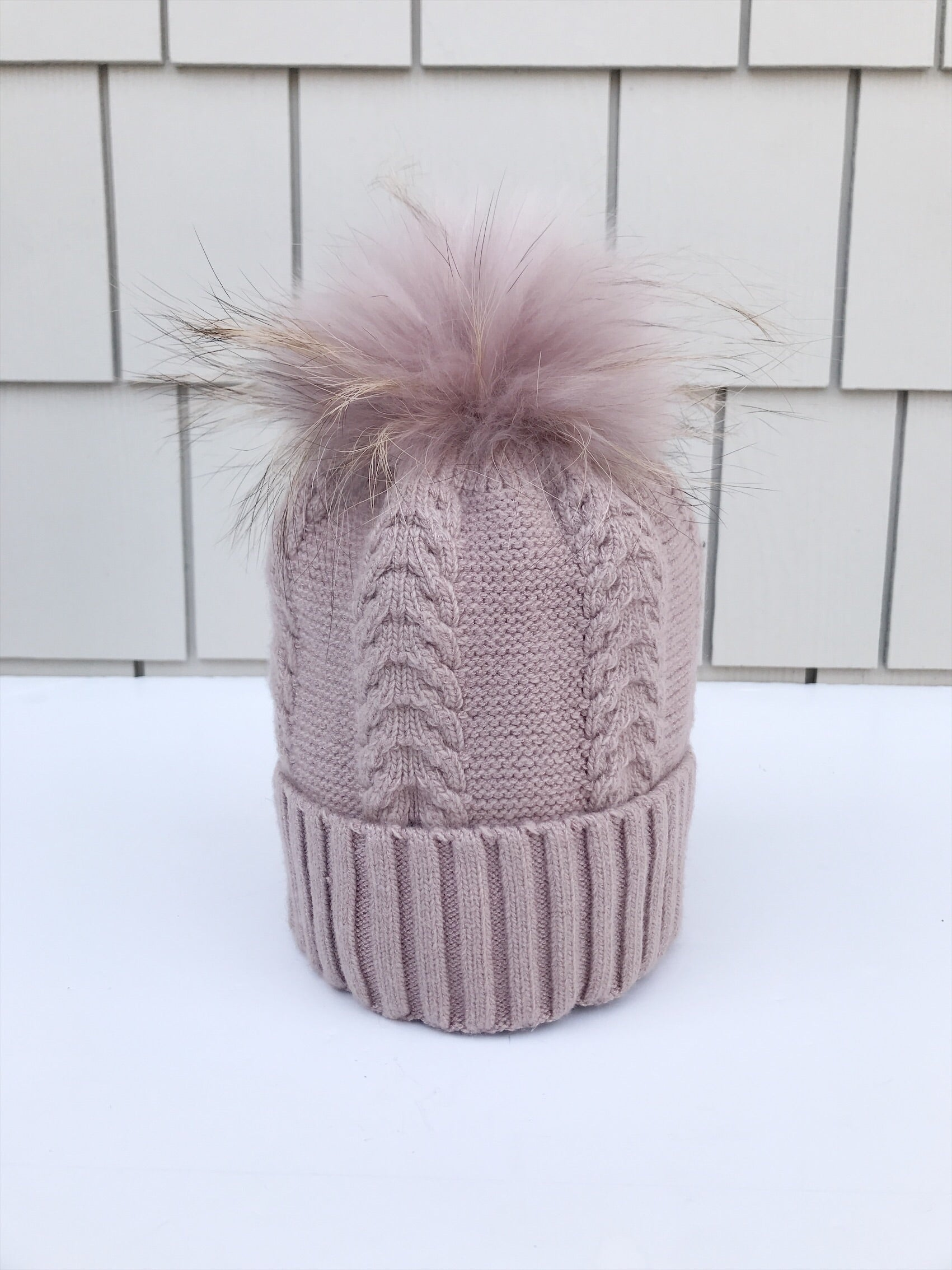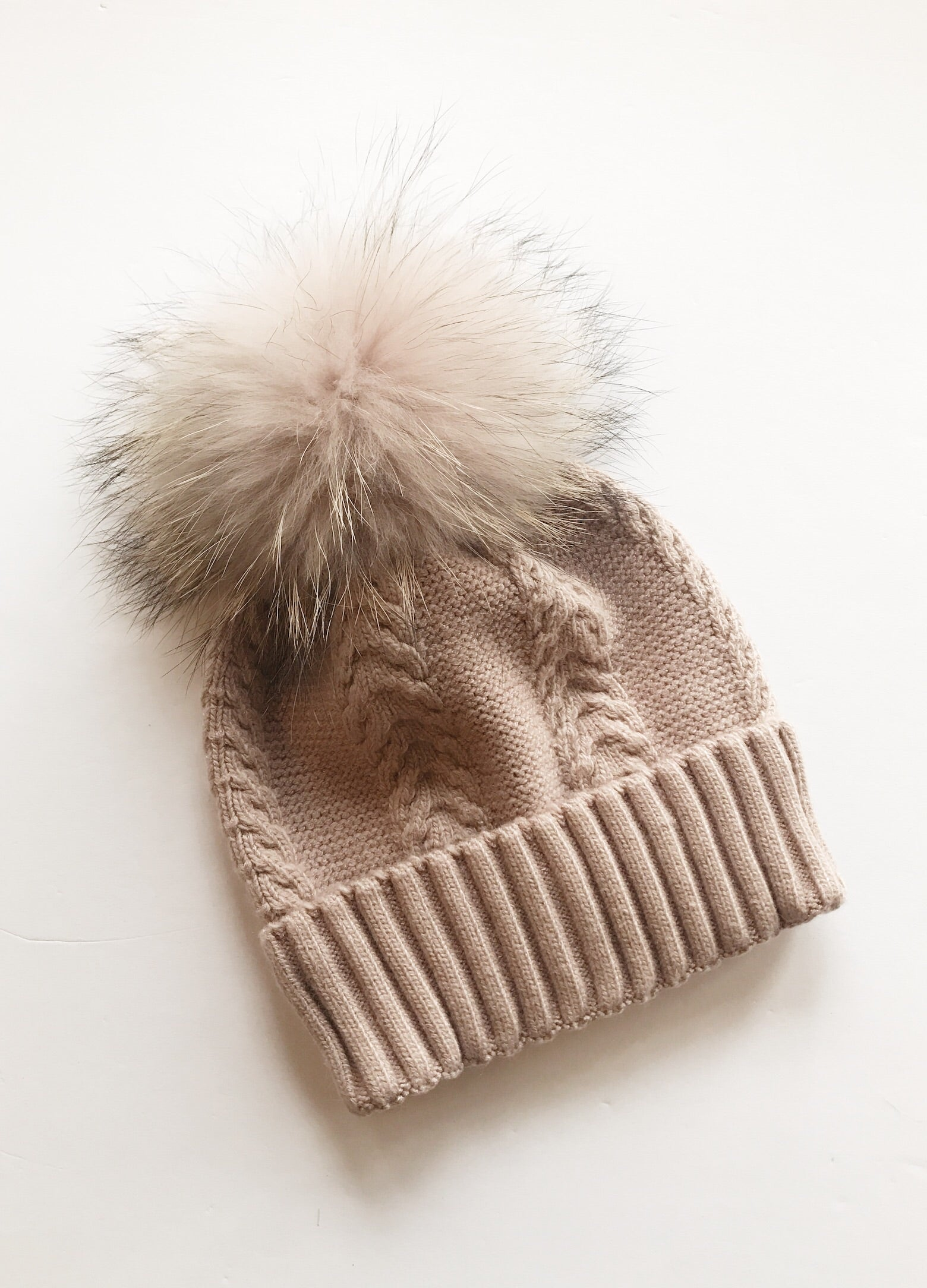Equation Olivia Hat in Pink w/ Pink pom
EQUATION
\$65.00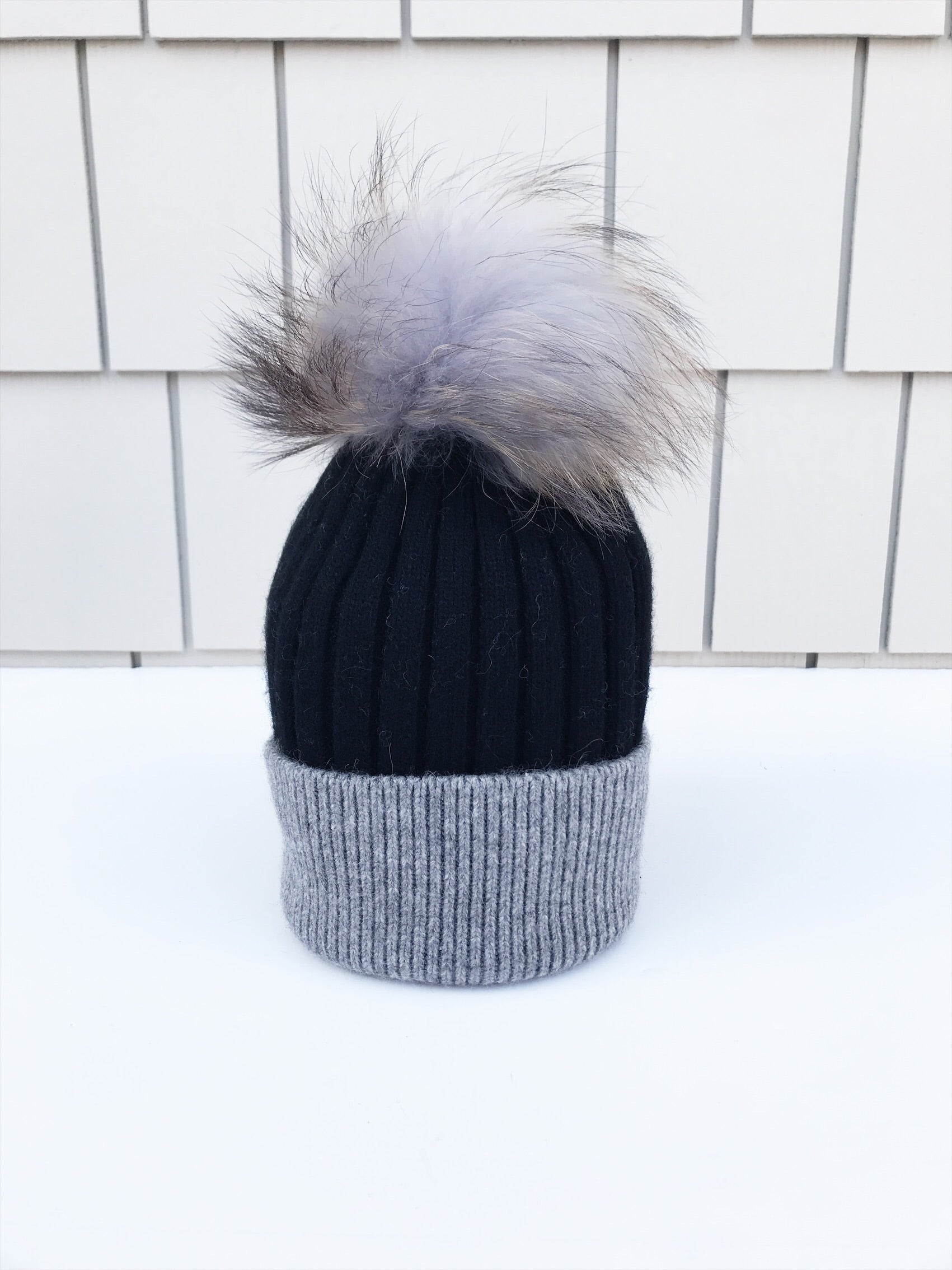Equation Milla Hat in Black/Gray
EQUATION
\$65.00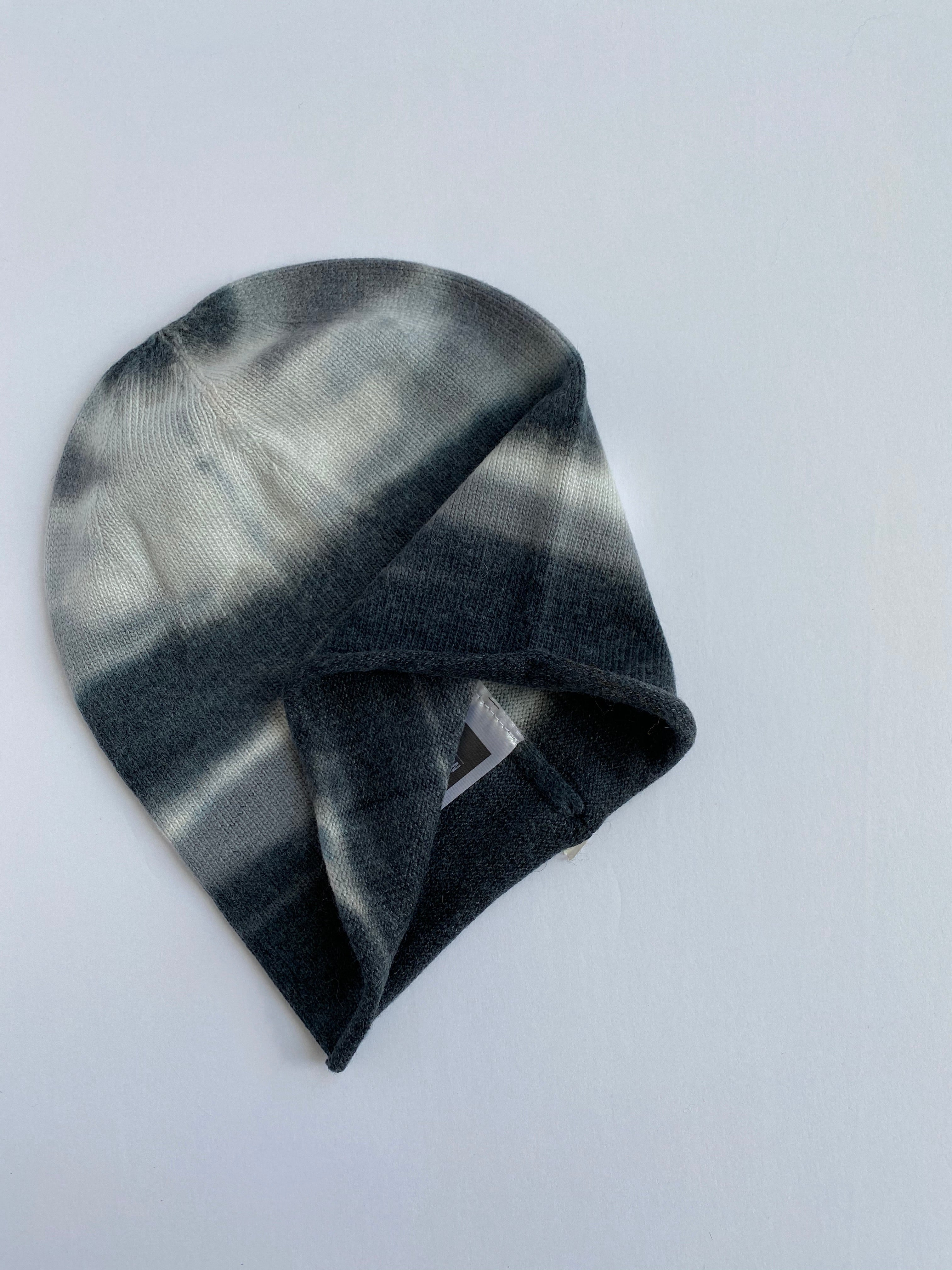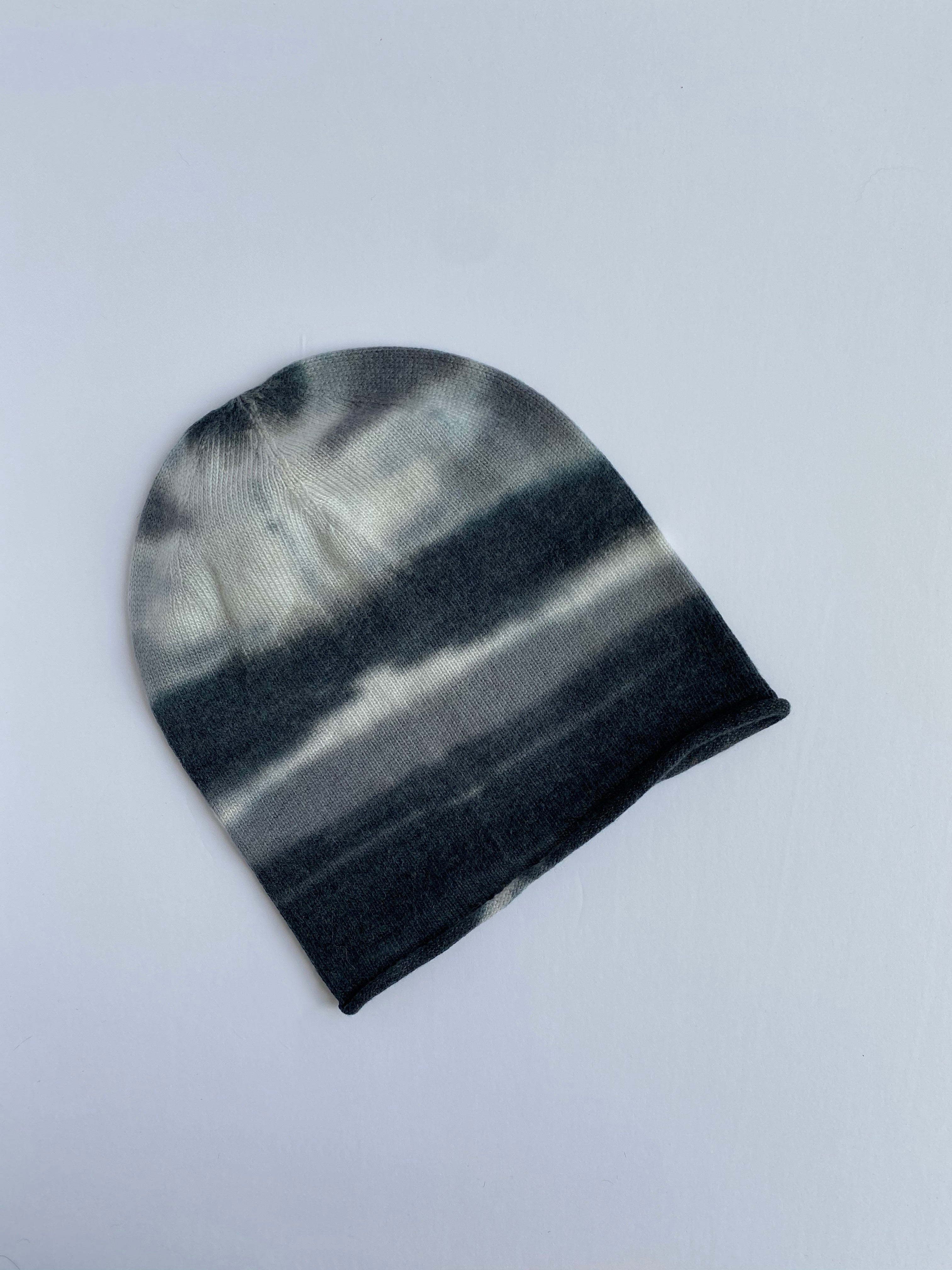Equation Chelsea Tie-dye Beanie Hat
EQUATION
\$48.00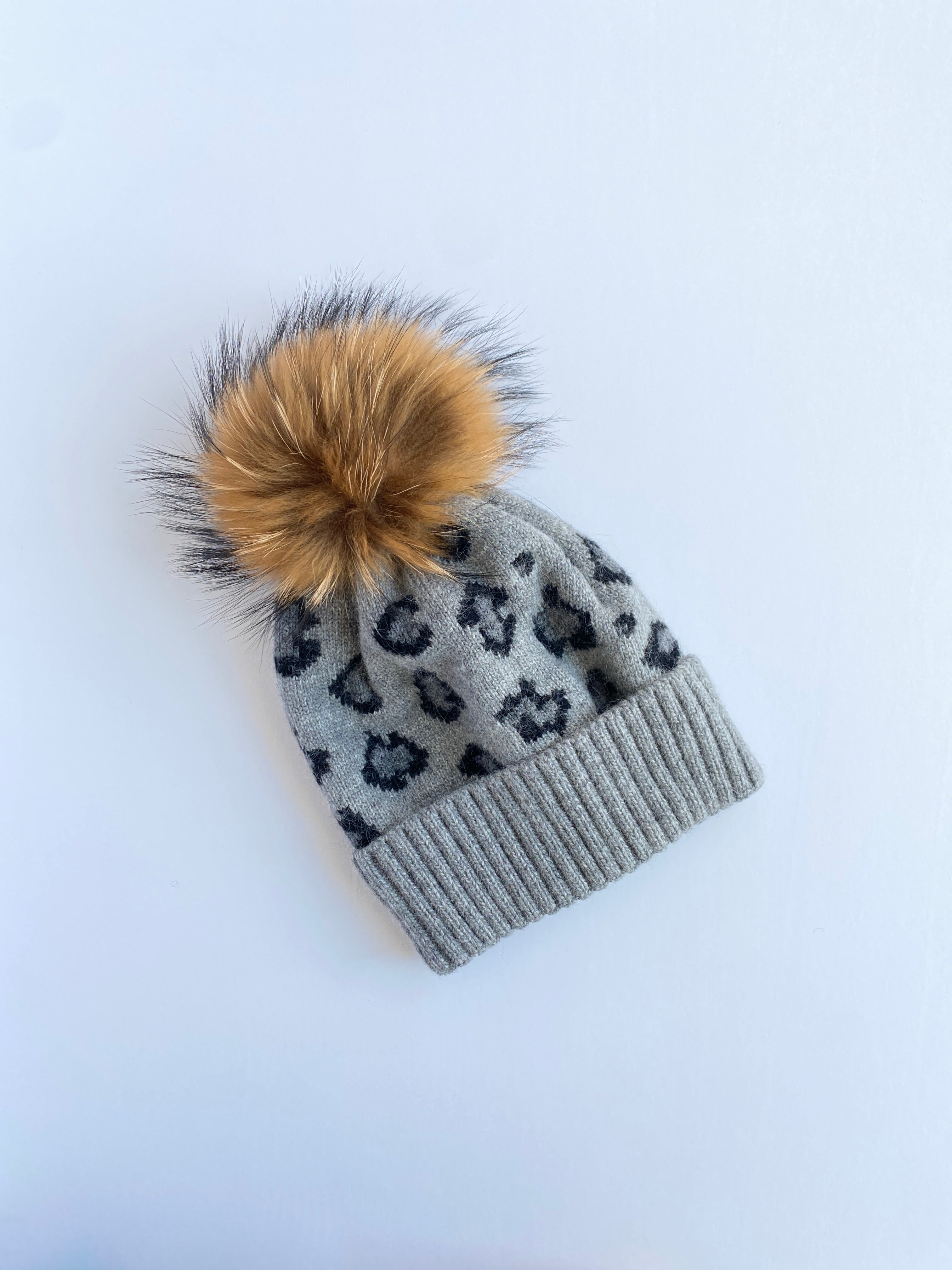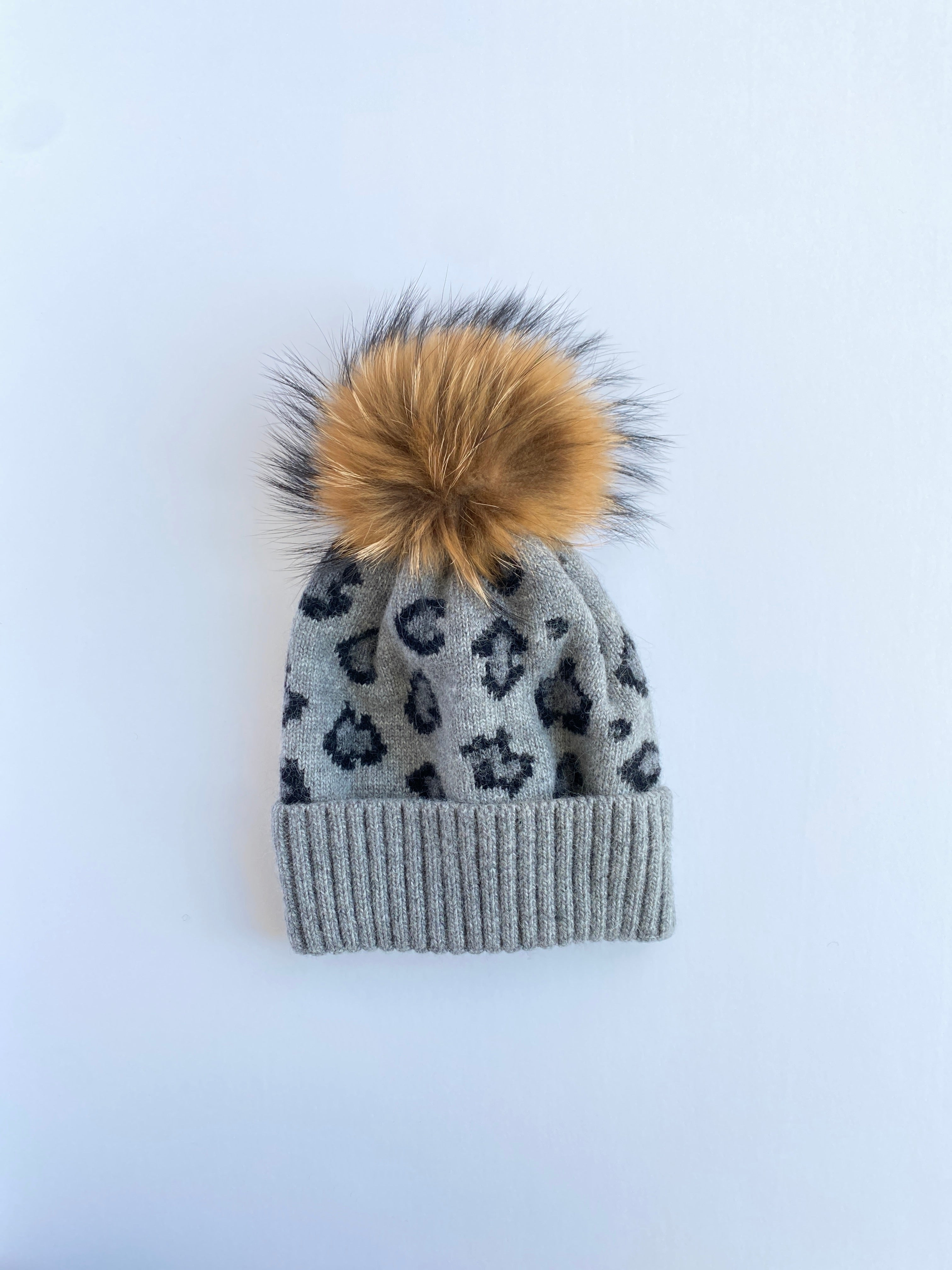Equation Leopard Hat in Gray
EQUATION
\$70.00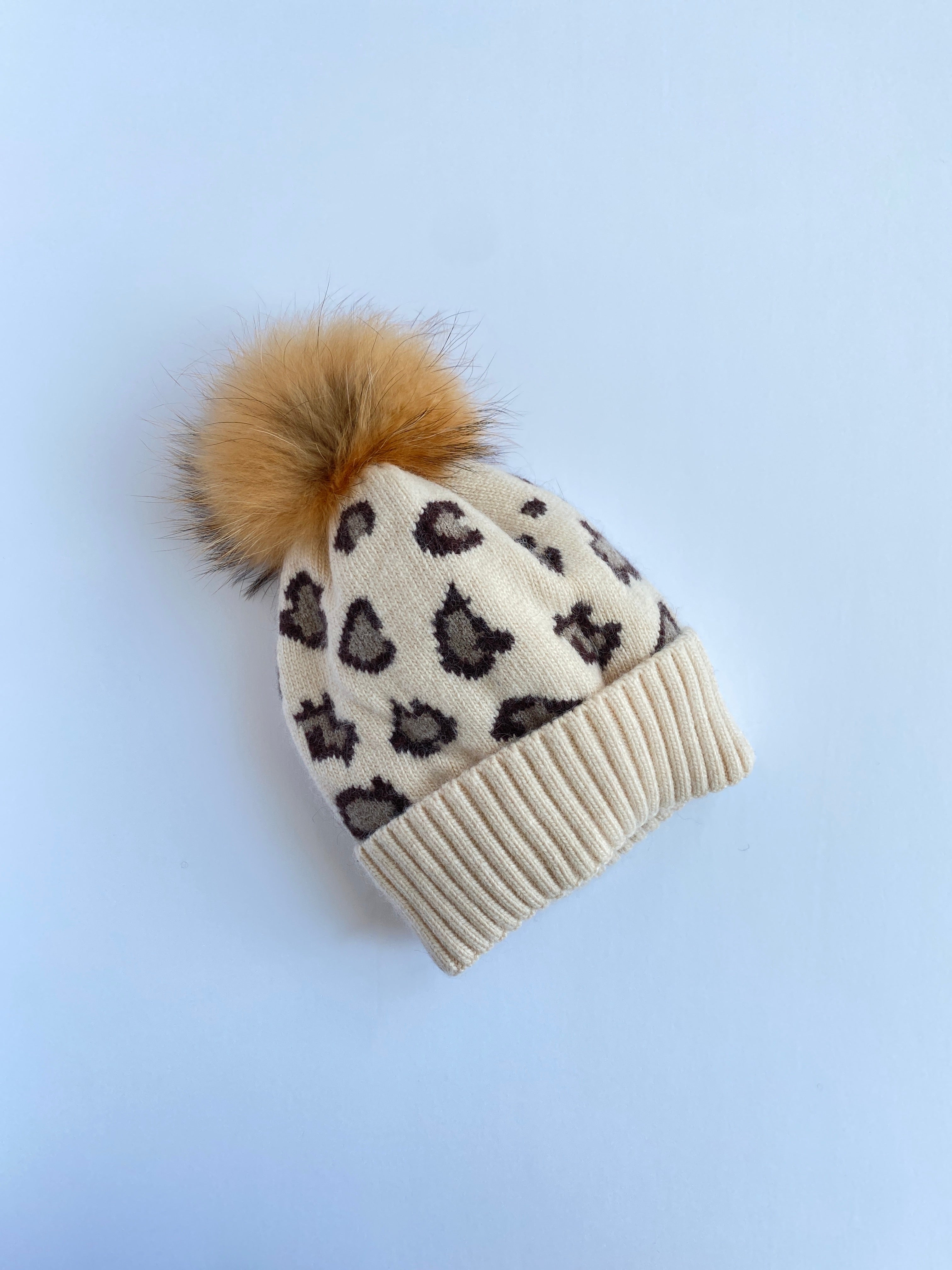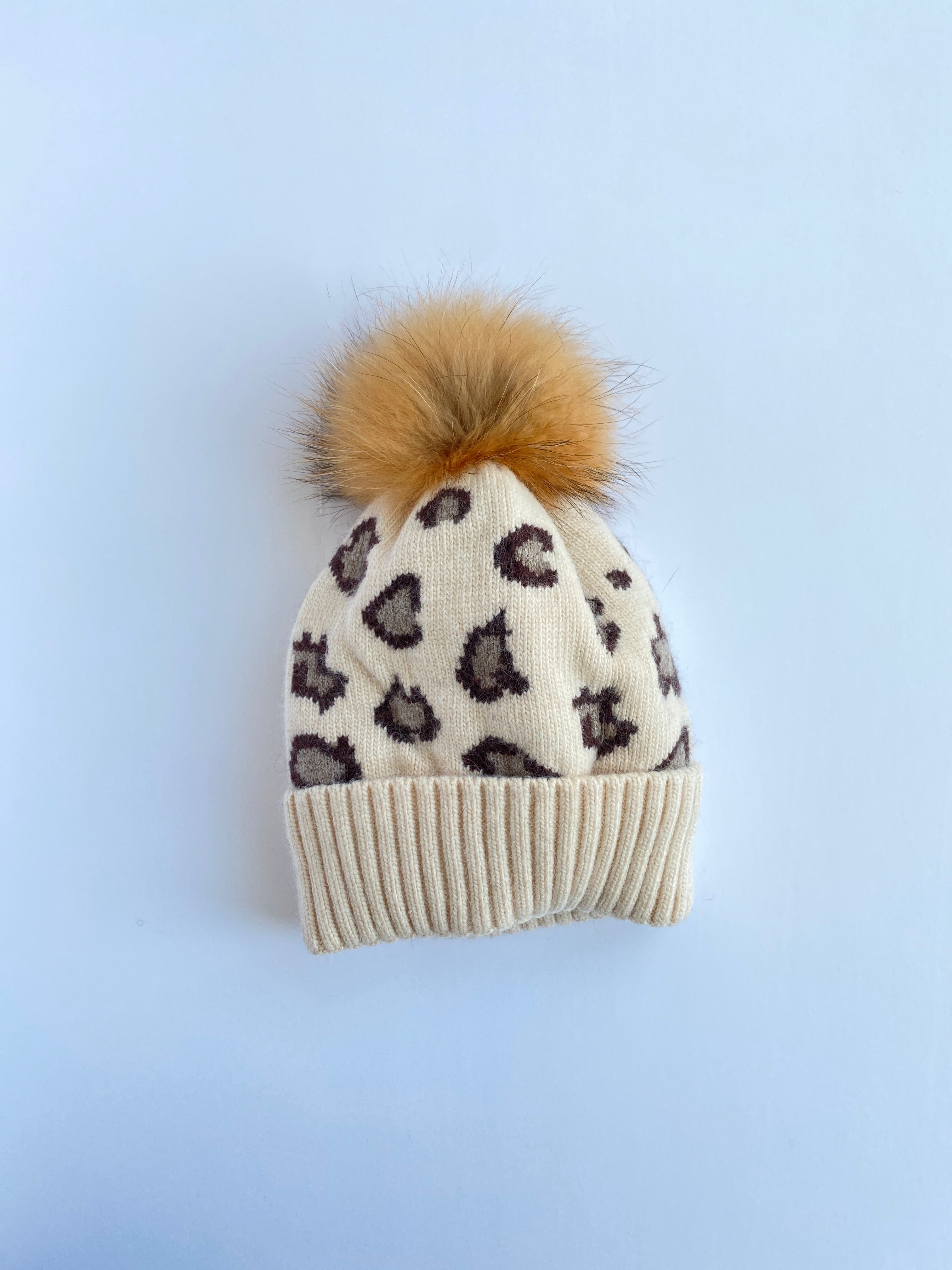Equation Leopard Hat in Cream
EQUATION
\$70.00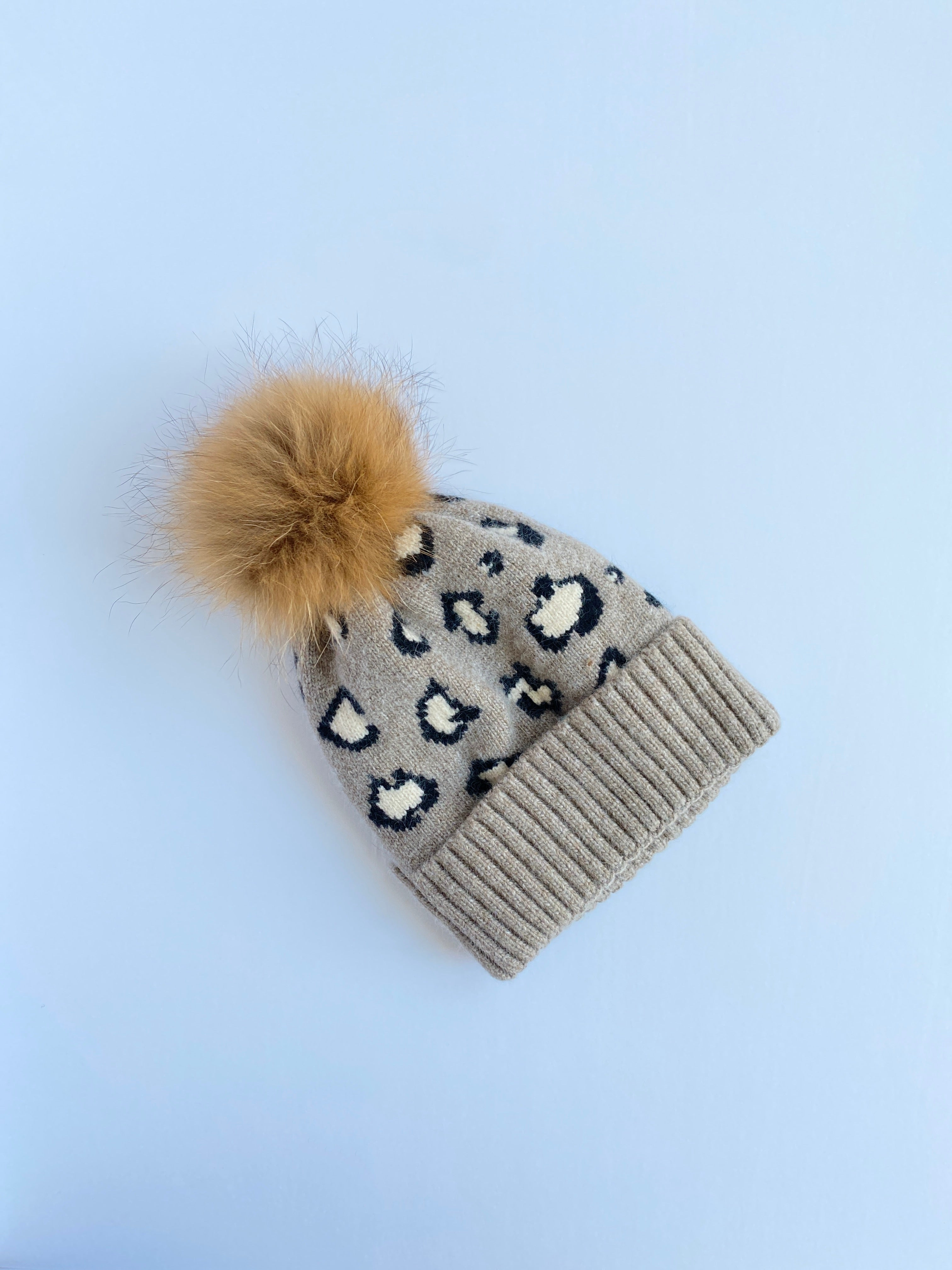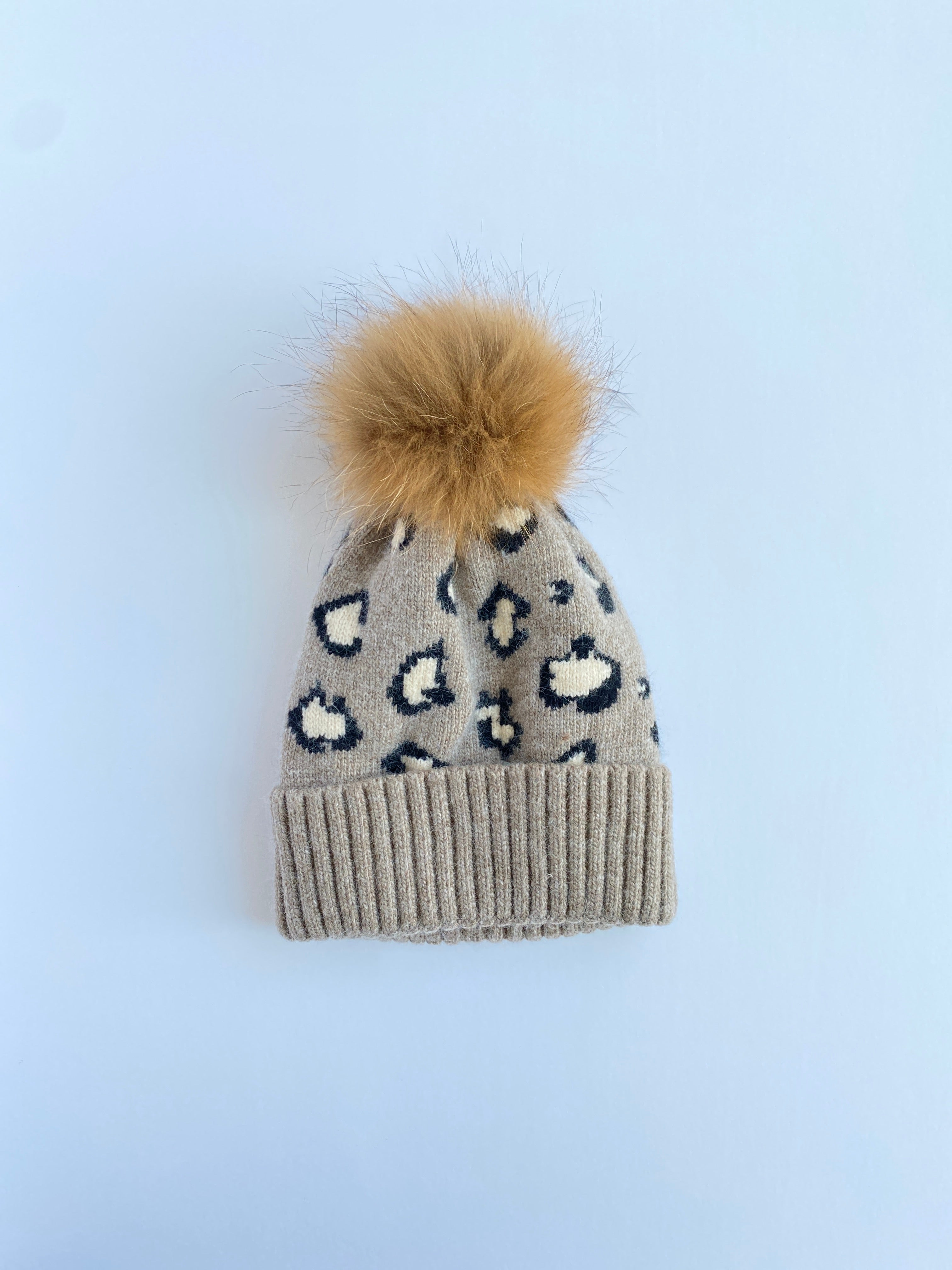Equation Leopard Hat in Oatmeal
EQUATION
\$70.00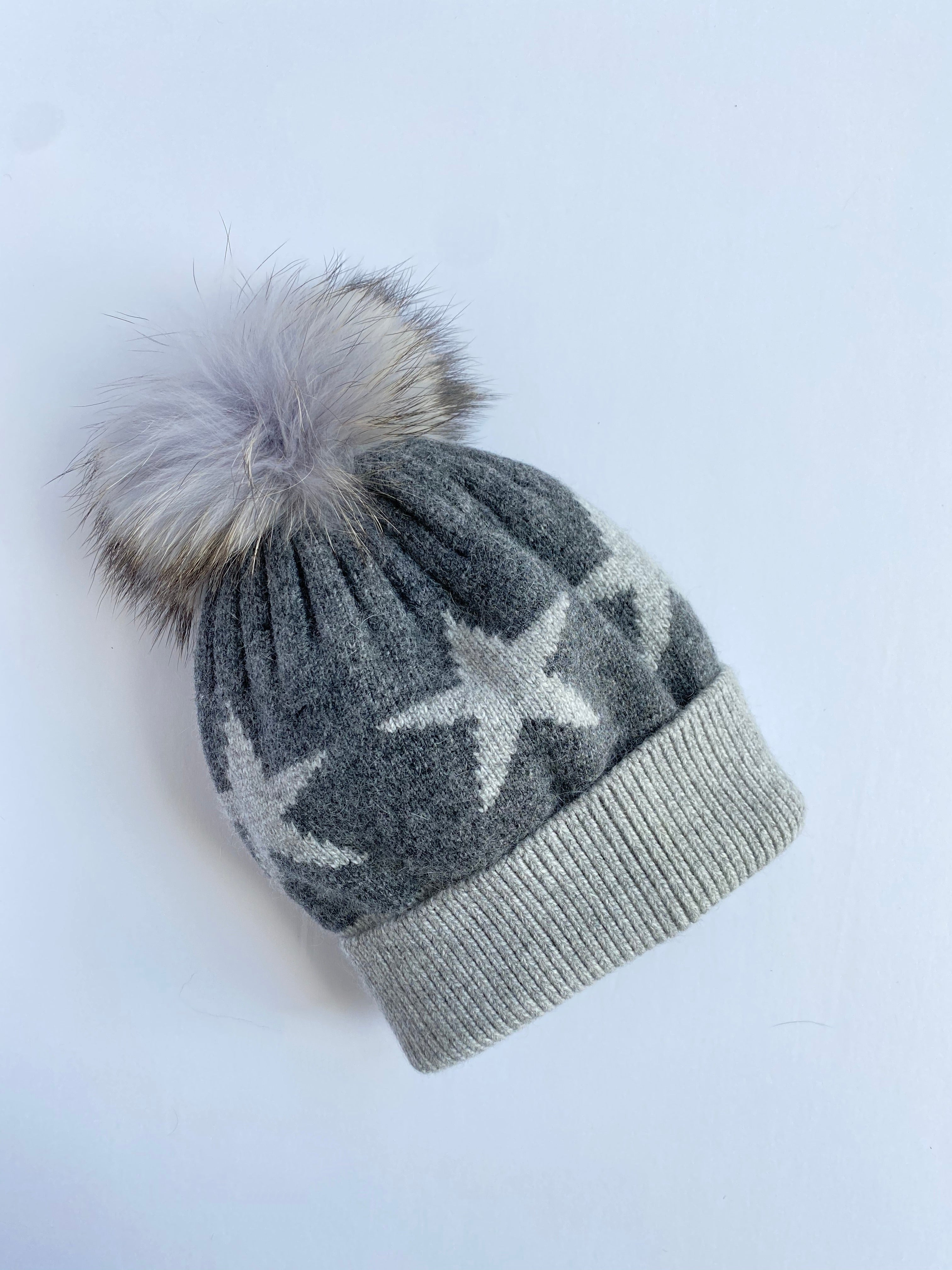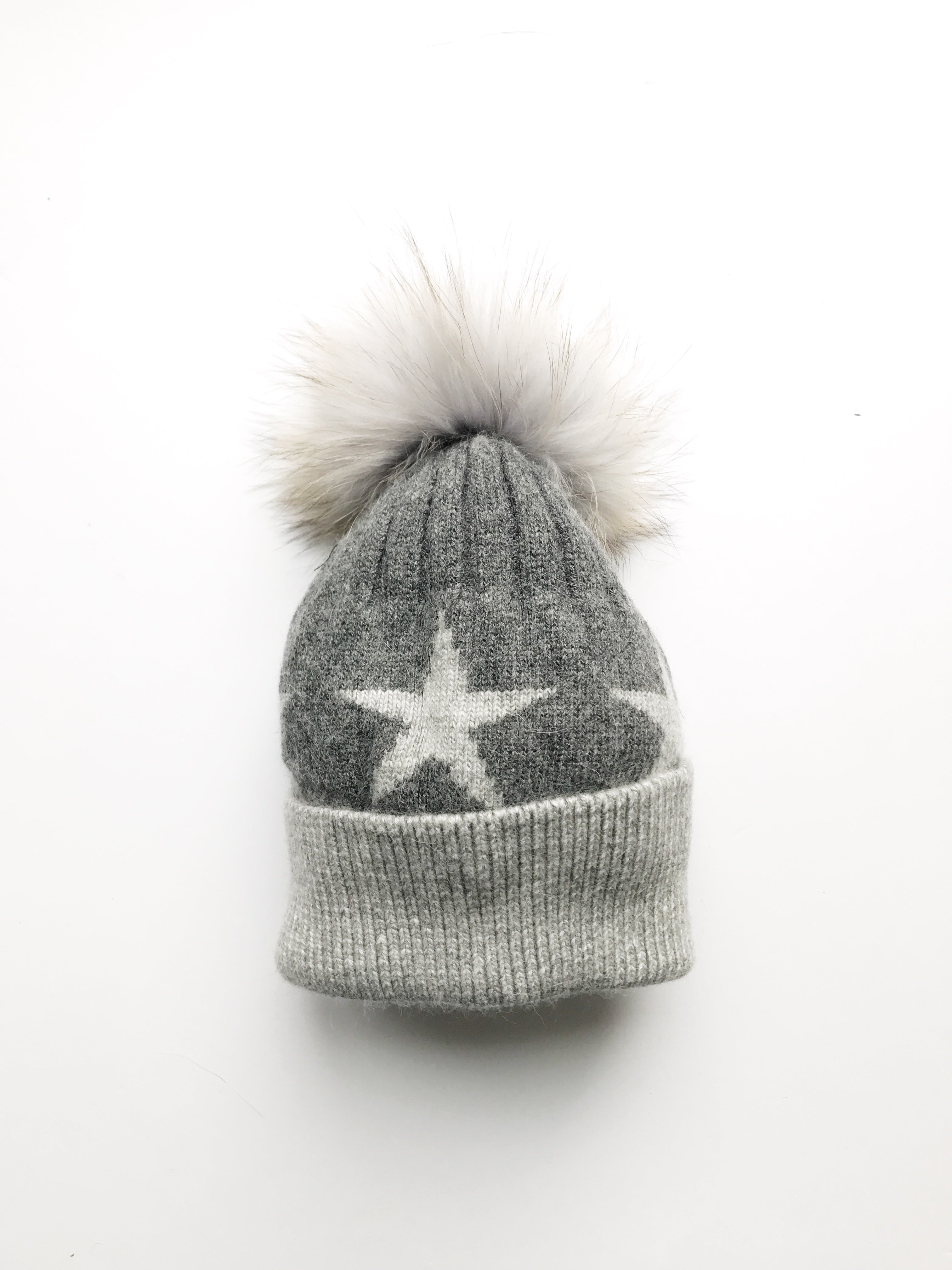Equation Starry Hat in Dark gray with heather gray stars
EQUATION
\$65.00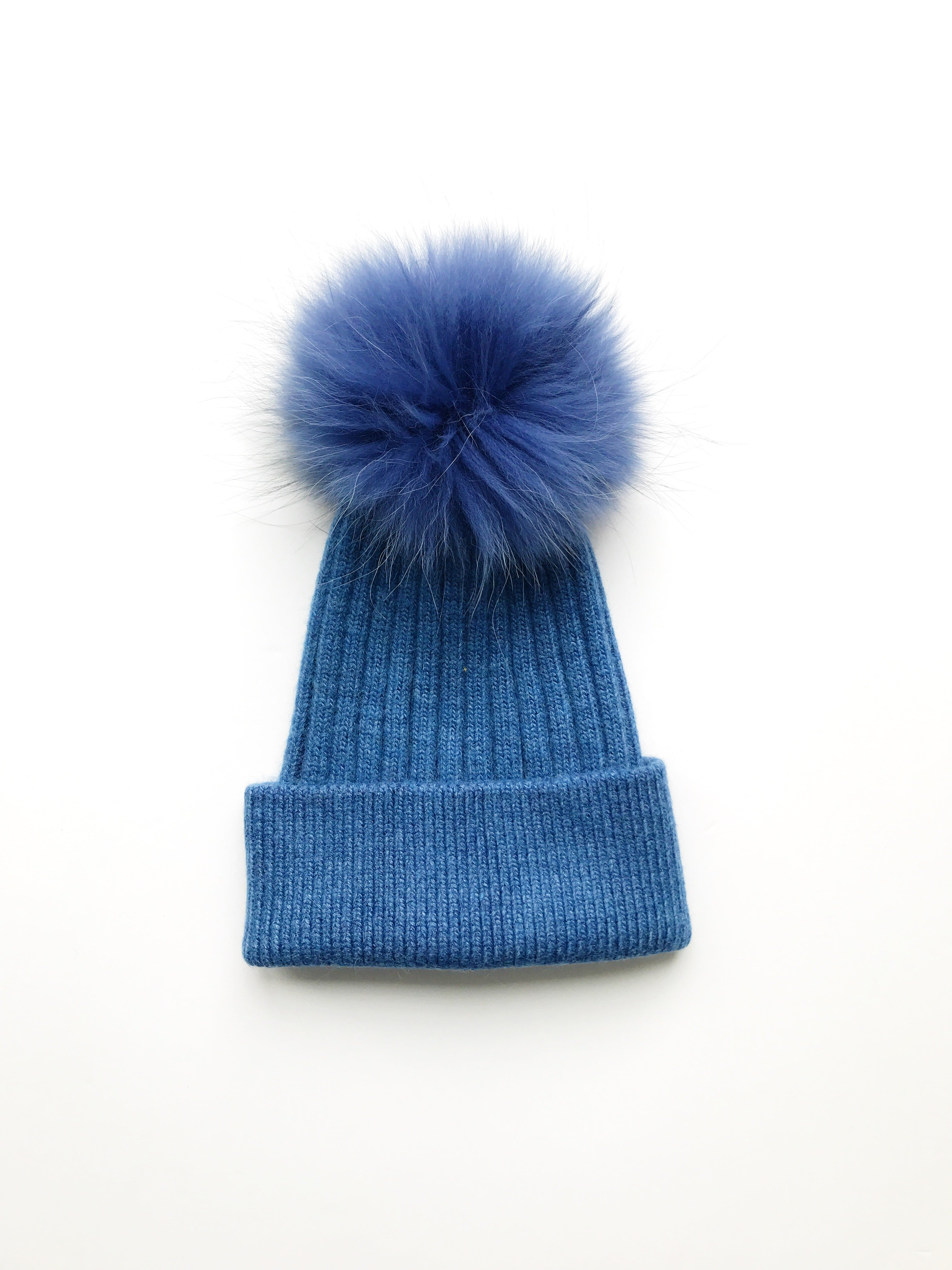Equation Elliott Hat in Denim w/ Denim pom
EQUATION
\$65.00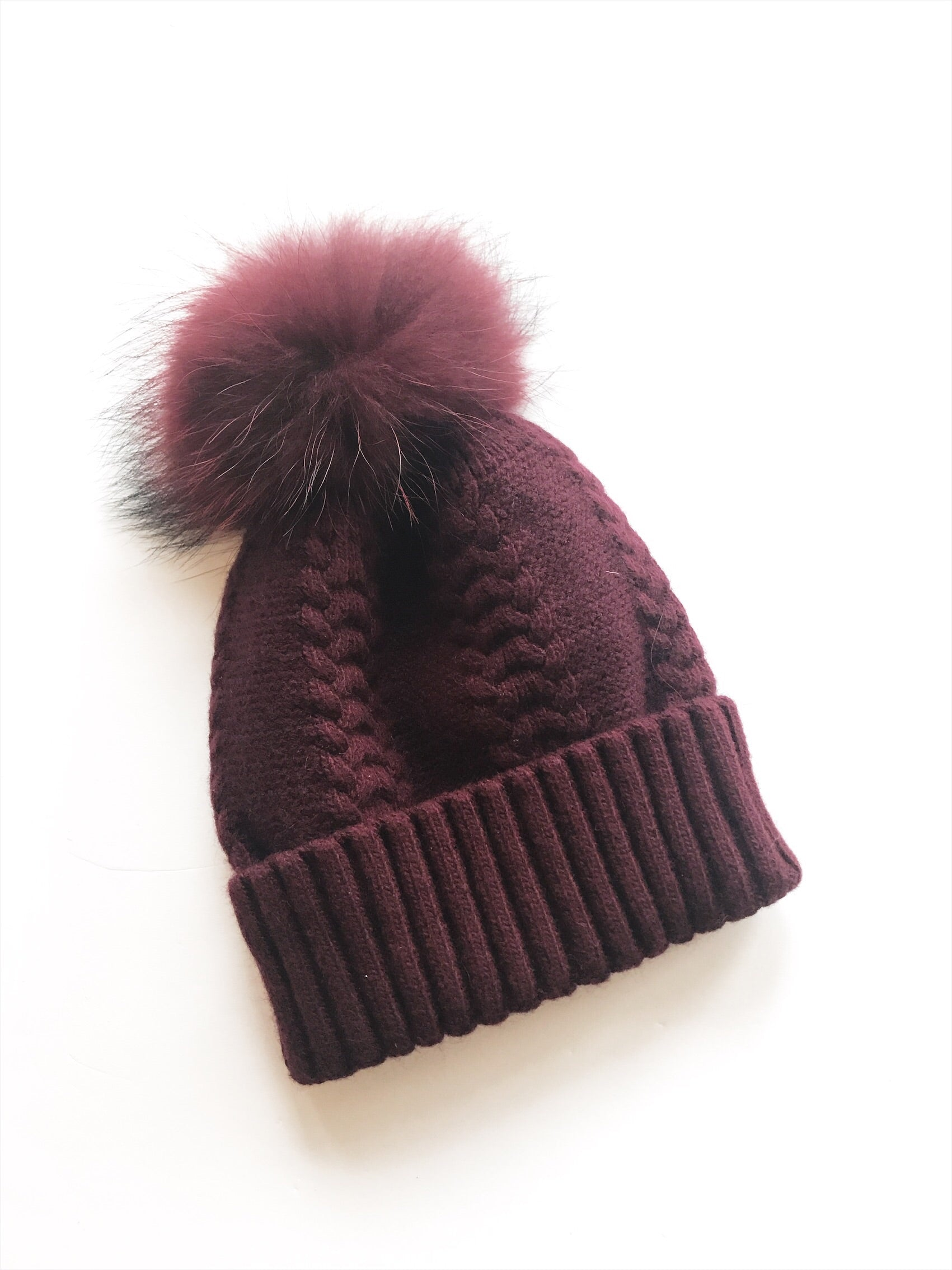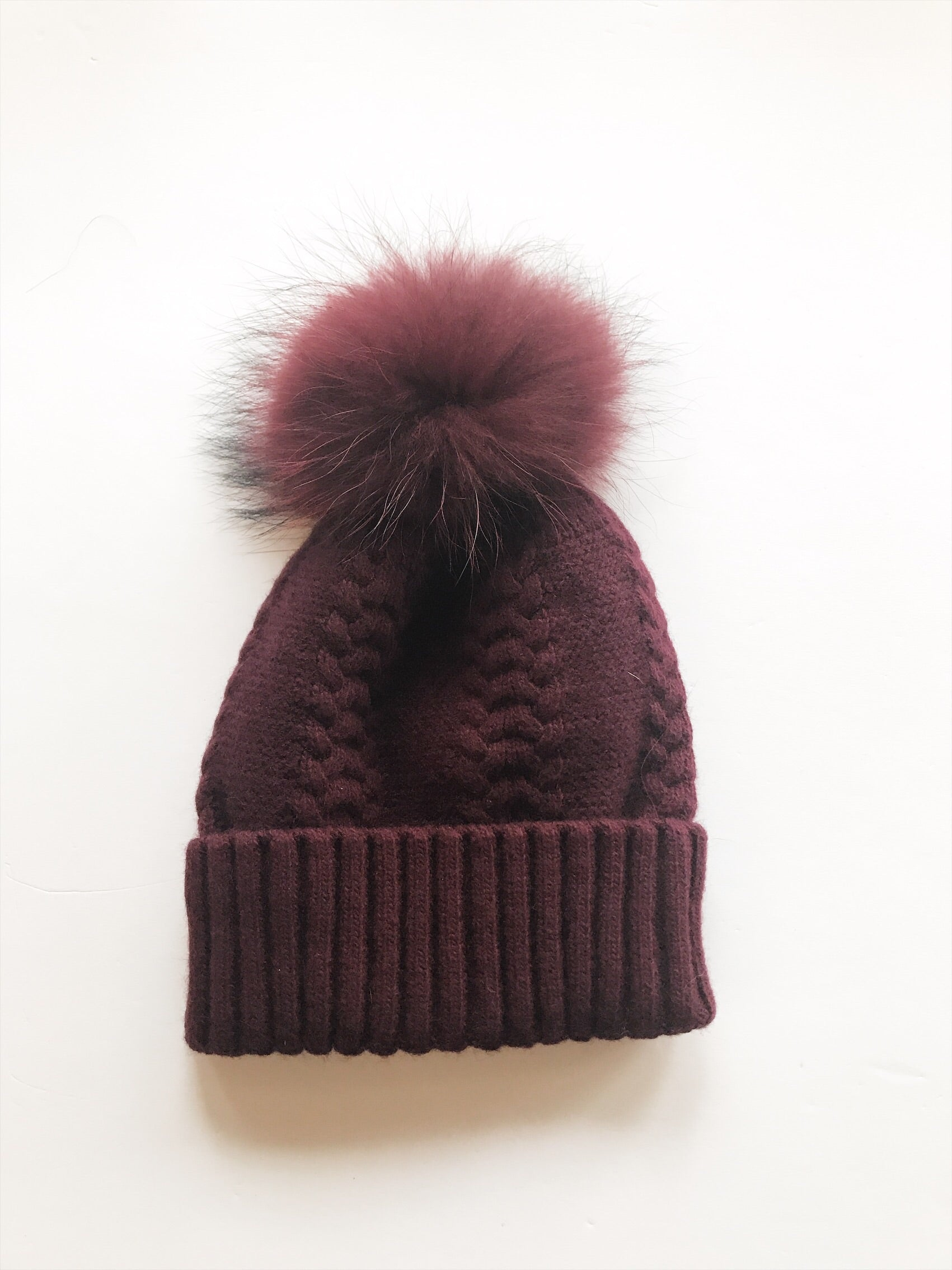Equation Olivia Hat in Wine w/ Wine pom
EQUATION
\$65.00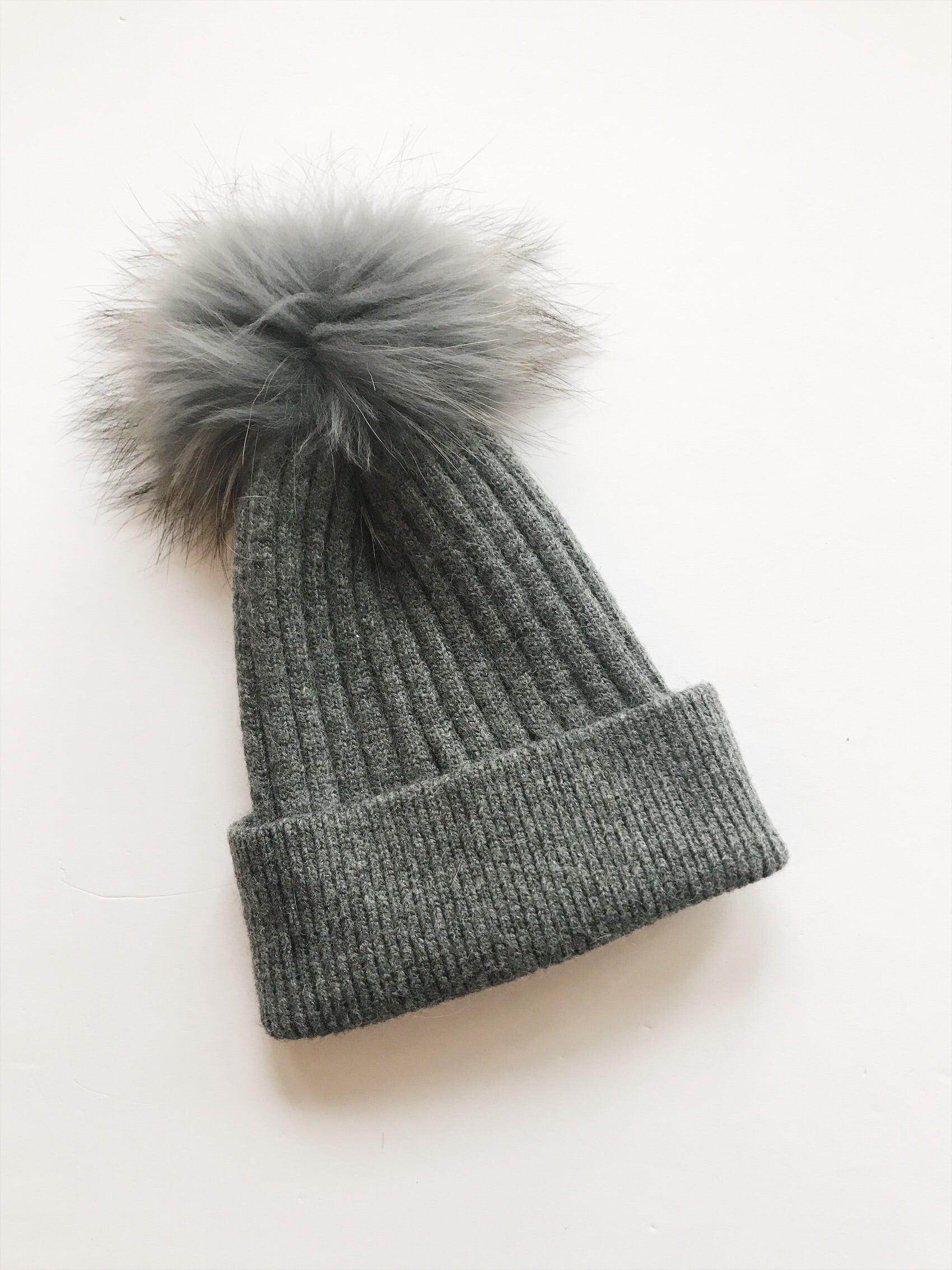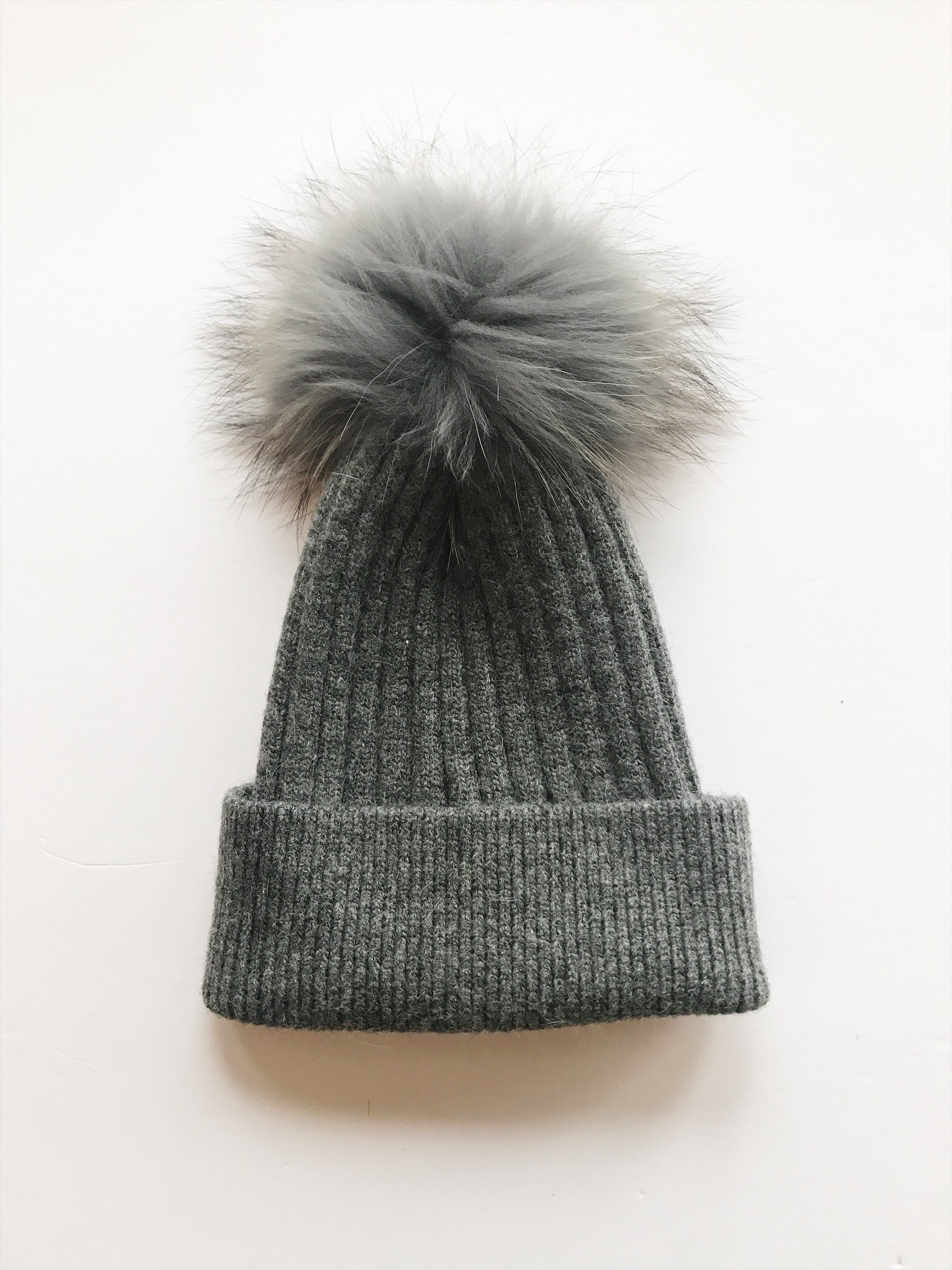Equation Elliott Hat in Dark gray w/ gray pom
EQUATION
\$65.00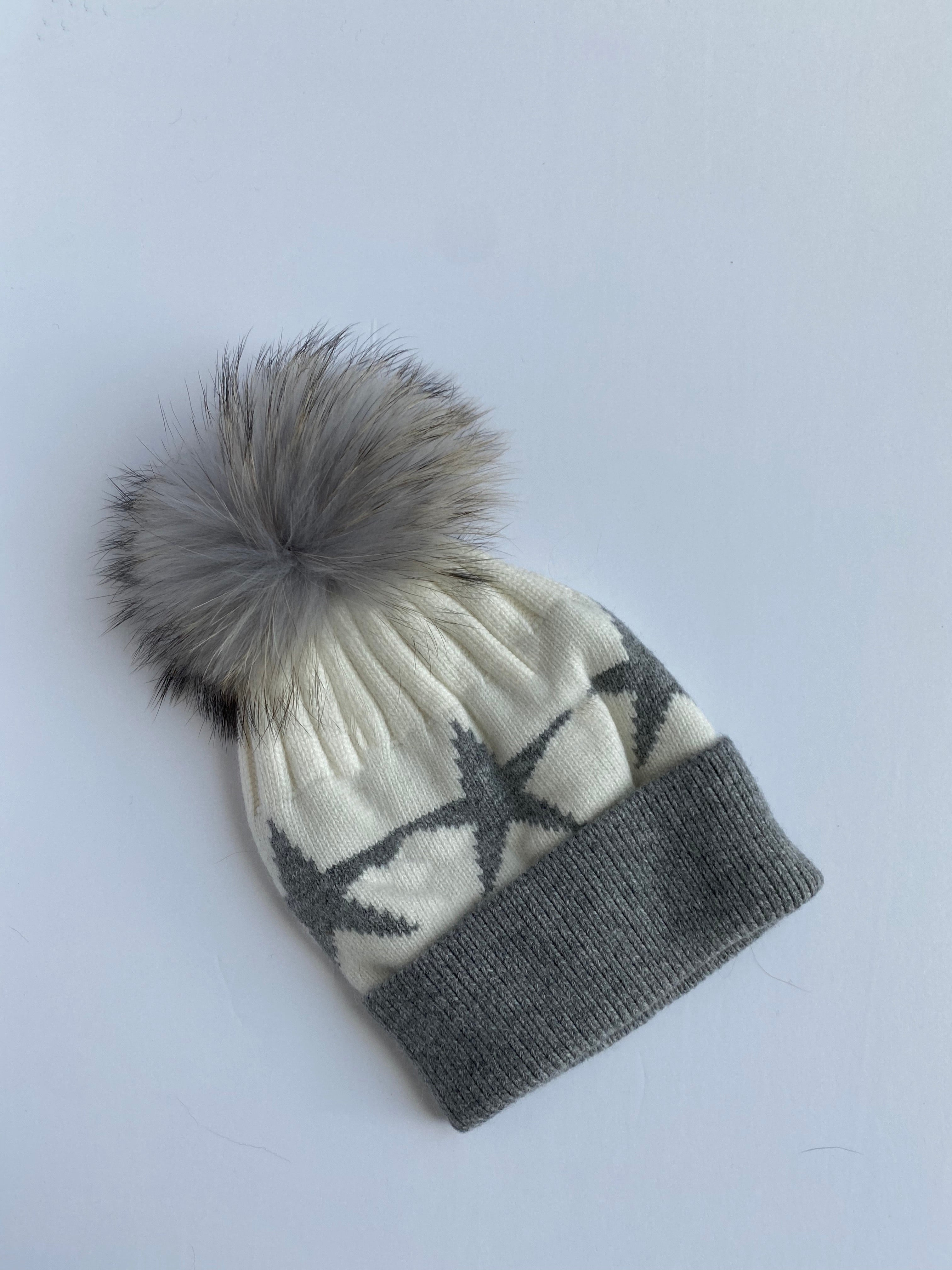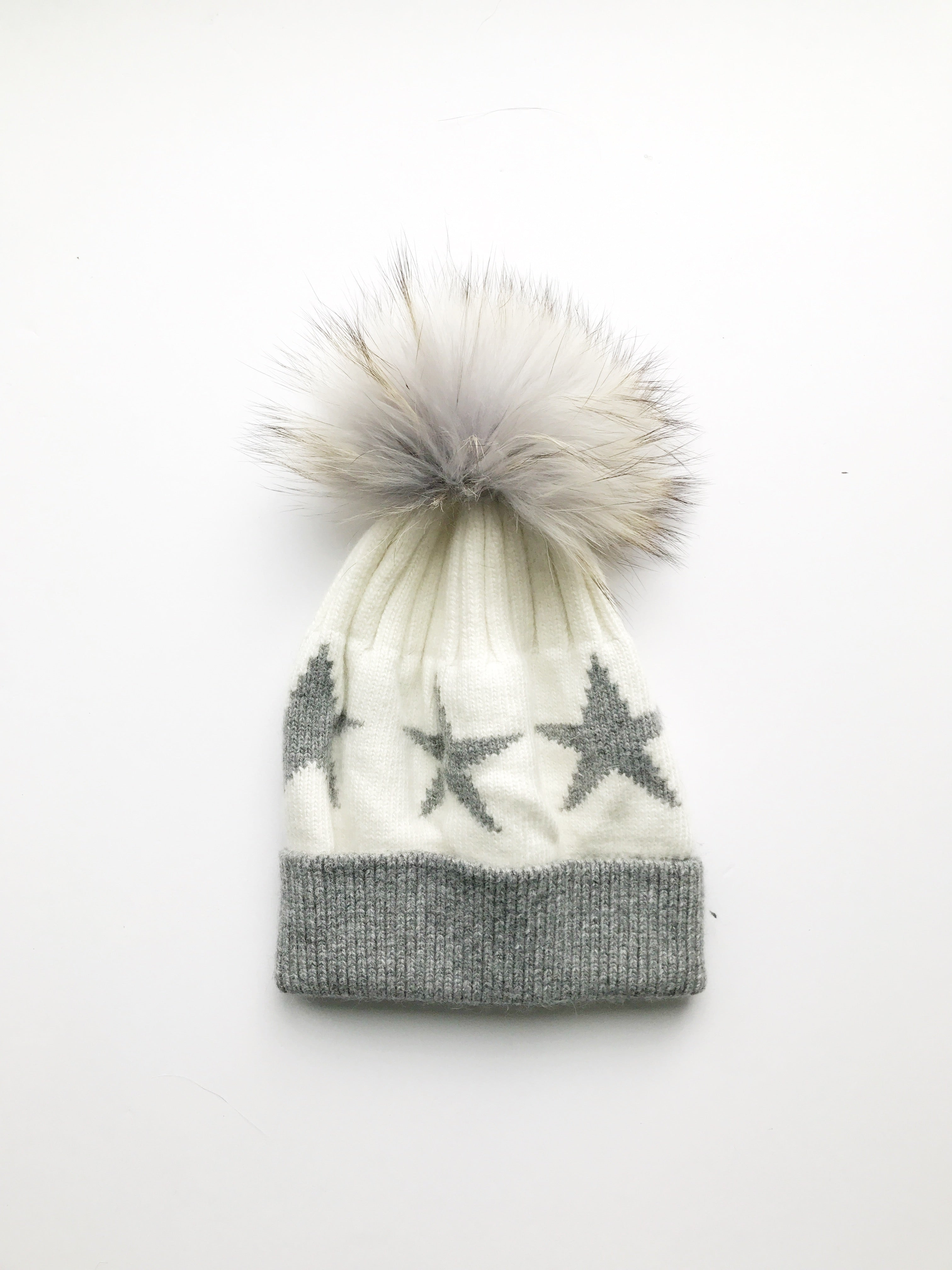Equation Starry Hat in White with heather gray stars
EQUATION
\$65.00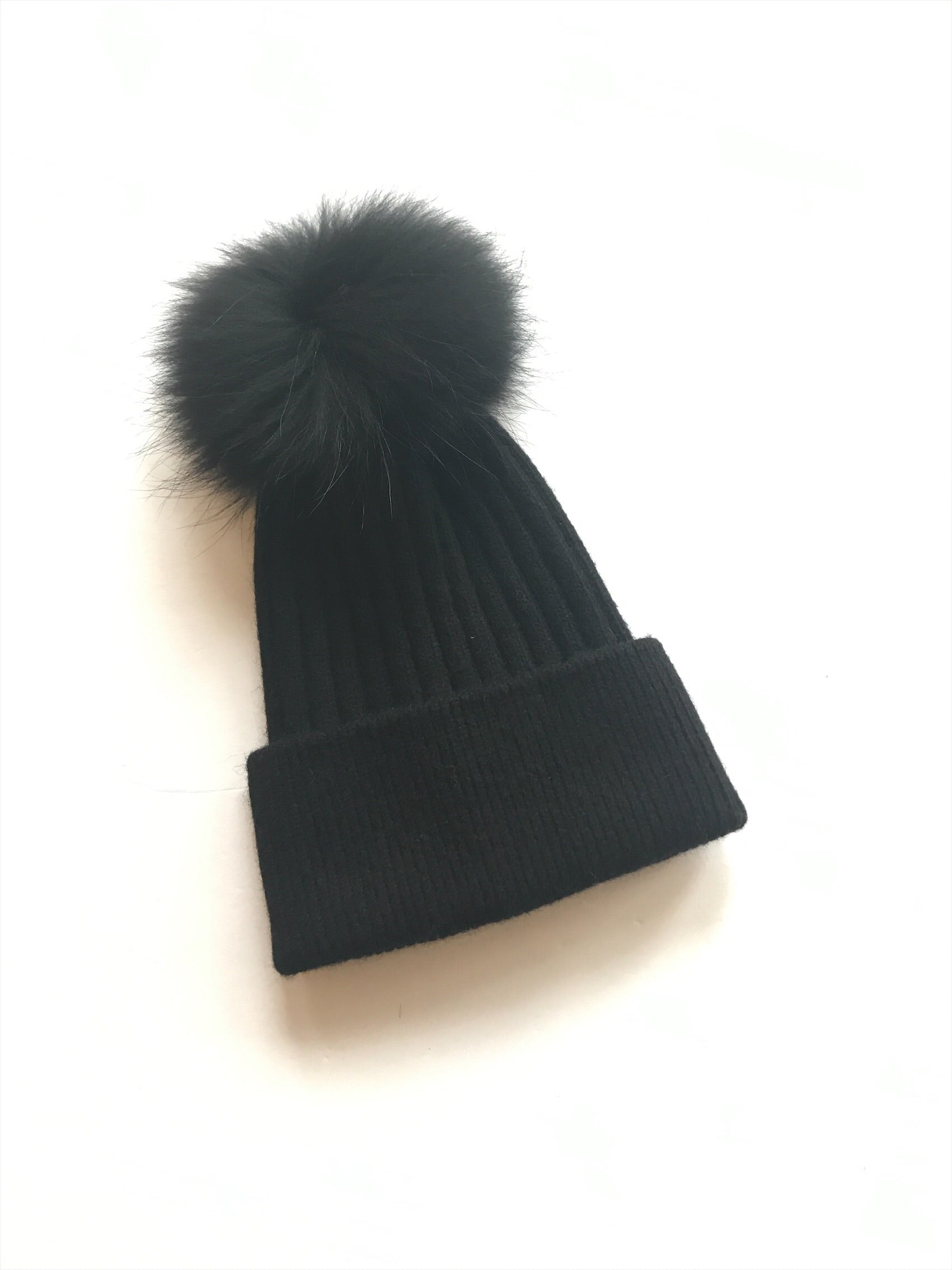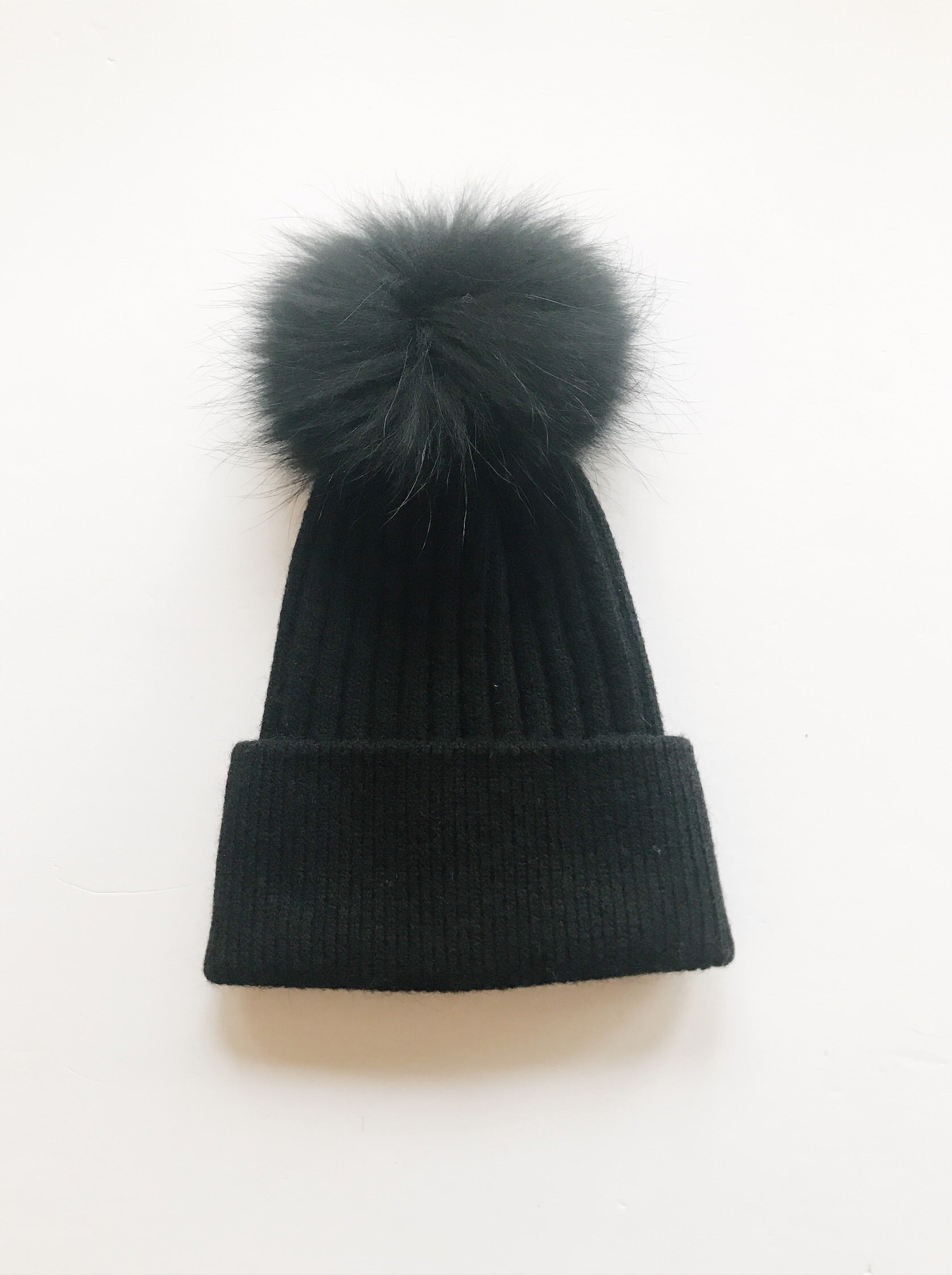Equation Elliott Hat in Navy w/ Navy pom
EQUATION
\$65.00